# Guide to nonlinear potential estimates

## Abstract

One of the basic achievements in nonlinear potential theory is that the typical linear pointwise estimates via fundamental solutions find a precise analog in the case of nonlinear equations. We give a comprehensive account of this fact and prove new unifying families of potential estimates. We also describe new fine properties of solutions to measure data problems.

## A synopsis

The aim of this paper is to give a rather comprehensive introduction to nonlinear potential estimates, i.e., pointwise estimates for solutions to quasilinear, possibly degenerate elliptic equations via linear and nonlinear potentials. The paper contains both new and old results. They fall into two categories. The first consists of those results that have been proved elsewhere, and that are here given in different and/or streamlined version. The second contains new results that are presented for the first time. These fill some of the gaps that were making the current theory still somewhat incomplete.

To ease the reading, the already known results will be stated together with the reference to the corresponding original paper where they have appeared for the first time. The new ones will be presented pointing at the place of this paper where the proof can be found. In general, the first part of the paper is devoted to the presentation of the general setting, with the statements of the main theorems; this goes up to Sect. 8. The remaining parts are instead devoted to the proofs.

We shall start from a presentation of the classical results valid for linear elliptic equations in Sect. 2, and this will serve to give the general guideline to the topics we are going to cover in the nonlinear case. The first potential estimates for nonlinear equations will be introduced in Sect. 3. There the classical pointwise estimates will be presented. In the subsequent Sects. 4 and 5 we shall instead give a class of estimates aimed at unifying the theory. These allow to frame the classical pointwise potential inequalities in a more general setting, allowing for estimates of both size and oscillations of solutions and their derivatives, including fractional derivates. At this stage we shall use “intermediate” Wolff and Riesz potentials and various fractional maximal operators. Especially, we prove Theorem 10, whose role is to unify estimates for $u$ and $D u$ via a family of estimates for suitable fractional operators of $u$. We shall eventually add a few remarks on the case of equations with coefficients in Sect. 6. All the results up to Sect. 6 are presented for energy solutions, that is for solutions belonging to the natural energy space $W 1 , p$ associated to the problems considered. In Sect. 7 we then turn to the case of general measure data problems, that is when very weak solutions come into the play. This will give us the opportunity, in Sect. 8, to present a few interesting consequences of potential estimates. Specifically, we shall prove two theorems about the possibility of describing the fine behaviour of solutions to nonlinear measure data problems and of their gradient via potentials. These theorems are the nonlinear analogs of classical results about fine properties of solutions to linear equations, which are usually derived via linear potential; see Remark 3 below. In Sect. 9 we then gather a series of regularity results that follow as a corollary of the theory presented. Some of these results are well-known and they are now framed in the general and unifying context of nonlinear potential estimates. The remaining sections are devoted to the proofs of the results introduced in the preceding ones, and their titles are self-explaining. In this paper we are not going to deal with parabolic problems, for which we refer to .

Before starting, we find useful to establish some notation. In what follows we denote by $c$ a general positive constant, possibly varying from line to line; special occurrences will be denoted by $c 1 , c 2 , c ¯ 1 , c ¯ 2$ or the like. All these constants will always be larger or equal than one; moreover relevant dependencies on parameters will be emphasized using parentheses, i.e., $c 1 ≡ c 1 ( n , p , ν , L )$ means that $c 1$ depends only on $n , p , ν , L$. We denote by

$B ( x , r ) ≡ B r ( x ) : = { x ~ ∈ R n : | x ~ - x | < r }$

the open ball with center $x$ and radius $r > 0$. When not important we shall omit denoting the center as follows: $B r ≡ B ( x , r )$. Moreover, with $B$ being a generic ball with radius $r$, we will denote by $σ B$ the ball concentric to $B$ having radius $σ r$, $σ > 0$. Unless otherwise stated, different balls in the same context will have the same center. The symbol $Ω$ will denote a bounded open subset of $R n$ and we shall always consider the case $n ≥ 2$. With $O ⊂ R n$ being a measurable subset with positive measure, and with $g : O → R n$, $n ≥ 1$, being a measurable map, we shall denote by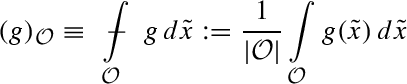its integral average; here $| O |$ denotes the Lebesgue measure of $O$. The map $g$ will be typically a gradient. We shall denote

$| g | ( O ) : = ∫ O | g | d x ~$

therefore adopting a unified notation for both measures and integrals of $L 1$-maps. In the following, $μ$ will always denote a Borel measure with finite total mass, which is initially defined on a certain open subset $Ω ⊂ R n$. Since this will not affect the rest, with no loss of generality, all such measures will be considered as defined in the whole $R n$ so that $| μ | ( R n ) < ∞ .$ We shall denote by $M b$ the space of all such measures.

## From linear to nonlinear

When considering linear elliptic equations, a powerful tool for establishing the qualitative properties of solutions is given by representation formulas via fundamental solutions. Eventually, these lead to consider linear Riesz potentials and singular integrals. Let us recall the situation for the simplest example, which is of course given by the classical Poisson equation

$- ▵ u = μ .$
(1)

For the sake of exposition, we initially consider the last equation in the whole $R n$, with $n ≥ 2$. Here $μ$, which is eventually taken to be a measure, is again for simplicity assumed to be a smooth and compactly supported function, while $u$ is the unique solution which decays to zero at infinity. The point we are interested in now is that $u$ can be recovered via convolution with the so called fundamental solution

$G ( x , y ) ≈ | x - y | 2 - n if n ≥ 3 - log | x - y | if n = 2$
(2)

and this means that the following representation formula holds:

$u ( x ) = ∫ R n G ( x , y ) d μ ( y ) .$
(3)

The formula in the last display allows to shift the study of solutions to the analysis of a related integral operator. It is therefore time for the following:

### Definition 1

(Riesz potentials) Let $α ∈ ( 0 , n ]$; the linear operator defined by

$I α ( μ ) ( x ) : = ∫ R n d μ ( x ~ ) | x - x ~ | n - α ,$
(4)

is called the $α$-Riesz potential of $μ$, where $μ$ is a Borel measure defined on $R n$.

Now, (3) allows to conclude with the following pointwise potential estimates:

$| u ( x ) | ≲ I 2 ( | μ | ) ( x ) | and | D u ( x ) | ≲ I 1 ( | μ | ) ( x ) .$
(5)

The first inequality actually holds in the case $n ≥ 3$; the second one follows differentiating (3). Since the behaviour of Riesz potentials with respect to various relevant function spaces is known (see for instance [1, 26]), using (5) allows to infer size properties of solutions from those of the relevant potentials, and in a sharp way. For instance, the following regularizing property of Riesz potentials is known for every $q > 1$ and $α > 0$ such that $0 < α q < n$:

$‖ I α ( μ ) ‖ L n q n - α q ( R n ) ≤ c ( n , α , q ) ‖ μ ‖ L q ( R n ) .$

As an immediate corollary, it follows for instance that $μ ∈ L q$ implies that $D u ∈ L n q / ( n - q )$ whenever $1 < q < n$. Moreover, estimates in further function spaces can be obtained as well, and these yield borderline regularity results. An instance is given by the following Lorentz spaces criterion:

$μ ∈ L ( n , 1 ) ⟹ I 1 ( | μ | ) ∈ L ∞ ⟹ D u ∈ L ∞ .$
(6)

We recall that the Lorentz space $L ( q , γ ) ( Ω )$, for $1 ≤ q < ∞$ and $γ > 0$, consists of those measurable maps $μ$ satisfying the condition

$∫ 0 ∞ λ q | { x ~ ∈ Ω : | μ ( x ~ ) | > λ } | γ / q d λ λ < ∞$
(7)

so that $μ ∈ L ( n , 1 )$ means in particular that

$∫ 0 ∞ | { x ~ ∈ Ω : | μ ( x ~ ) | > λ } | 1 / n d λ < ∞ .$
(8)

A point, which is less emphasised concerning estimates (5) is that (3) also allows to bound oscillations of the solution $u$ in terms of suitable Riesz potentials. This point actually turns out of be of primary interest for us here. To see this, by using the elementary inequality

$| x - x ~ | 2 - n - | y - x ~ | 2 - n ≤ c ( n ) | x - x ~ | 2 - n - α + | y - x ~ | 2 - n - α | x - y | α ,$

which is valid whenever $x , y , x ~ ∈ R n$, we get the new representation formula

$| u ( x ) - u ( y ) | ≤ c I 2 - α ( | μ | ) ( x ) + I 2 - α ( | μ | ) ( y ) | x - y | α , 0 ≤ α ≤ 1 .$
(9)

The previous estimate allows to infer, again via potentials, information on the solutions in function spaces measuring smoothness rather than size. For instance, the Hölder continuity criterion

$I 2 - α ( | μ | ) ∈ L ∞ ⟹ u ∈ C 0 , α$

is immediate. Again, prescribing that $I 2 - α ( | μ | ) ∈ L q$ implies that $u$ belongs to the so called Calderón space $C q α$; see Definition 2 below, and [17, 40], for a discussion. In a similar way one gets estimates for the gradient

$| D u ( x ) - D u ( y ) | ≤ c I 1 - α ( | μ | ) ( x ) + I 1 - α ( | μ | ) ( y ) | x - y | α , 0 ≤ α < 1 .$
(10)

As a matter of fact, formulas (9)–(10) can be read as nonlocal, fractional derivatives versions of the classical estimates in (5). This is clarified by the following definition:

### Definition 2

(Calderón spaces) Let $α ∈ ( 0 , 1 ]$, $q ≥ 1$, and let $Ω ⊂ R n$ be an open subset. A measurable function $v$, finite a.e. in $Ω$, belongs to the Calderón space $C q α ( Ω )$ if and only if there exists a nonnegative function $m ∈ L q ( Ω )$ such that

$| v ( x ) - v ( y ) | ≤ [ m ( x ) + m ( y ) ] | x - y | α$
(11)

holds for almost every couple $( x , y ) ∈ Ω × Ω$.

This is just another way to say that $v$ has “fractional derivatives”. The advantage is that nonlocality is reduced to a minimal status: only two points are considered in (11). Moreover, when $Ω$ is a suitably regular domain, Calderón spaces are closely related to the usual fractional Sobolev spaces $W α , q$; see . The function $m$ plays in fact the role of a fractional derivative of $v$ of order $α$ in the $L q$-sense. Definition 2 is implicit in the work of DeVore and Sharpley , where the authors fix the canonical choice $m = M α # ( v )$ that is indeed always possible in (11) (see Proposition 1 below). The symbol $M α # ( v )$ denotes the standard fractional sharp maximal operator introduced in the following (take $Ω = R n$ and $R = ∞$):

### Definition 3

(Fractional sharp maximal operator) Let $β ∈ [ 0 , 1 ]$, $x ∈ Ω$ and $R ≤ dist ( x , ∂ Ω )$, and let $f ∈ L 1 ( Ω )$; the function defined byis called the restricted (centered) sharp fractional maximal function of $f$.

Notice that for $β = 0$ the previous definition gives back the classical sharp maximal operator of Fefferman and Stein (in the restricted version), which is in fact denoted by $M R # ( f )$. Definition 2 and the interpretation of $m$ as the $α$-order fractional derivative of $v$ allow to read the inequalities in (9)–(10) as a way to bound fractional derivatives of $u$ via intermediate Riesz potentials. We may express this concept in the following imprecise yet suggestive way:

$| ∂ α u ( x ) | ≲ I 2 - α ( | μ | ) ( x ) , 0 ≤ α < 2 .$
(12)

Needless to say, that last formula has only a symbolic meaning. At this point it is clear that the classical estimates (5) embed in the family of interpolating estimates (12) as particular borderline cases corresponding to the choices $α = 0$ and $α = 1$, respectively. In turn, the estimates in (12) provides a unifying approach to the regularity of solutions to (1). They allow to get results both in spaces aimed at measuring the size of functions and in those measuring smoothness.

One of the aims of this paper is to show that completely similar estimates actually hold for a large class of quasilinear, possibly degenerate equations. A potential theory which is completely analogous to the linear one can be constructed in the nonlinear case too.

## Basic nonlinear estimates

The main question is now whether and in which sense estimates like (5) and (12) extend to the case of solutions to quasilinear elliptic equations of the type

$- div a ( D u ) = μ in Ω .$
(13)

In the most general case, the right hand side $μ$ is a Borel measure with finite total mass in $R n$, i.e. $μ ∈ M b$. The one in (13) is a nonlinear version of the Poisson equation when the assumptions

$ν Id ≤ ∂ a ( z ) ≤ L Id$
(14)

are considered. The numbers $0 < ν ≤ L$ provide bounds for the lowest and highest eigenvalue of the matrix $∂ a ( · )$, respectively ($ν = L = 1$ gives the case of the Laplacean operator). We also want to consider cases where Eq. (13) might be degenerate, thereby examining for instance the so called $p$-Laplacean operator:

$- div ( | D u | p - 2 D u ) = μ .$
(15)

In order to catch the essential properties of the equation in (15), we shall consider general vector fields $a : R n → R n$. These are assumed to be $C 1$-regular and to satisfy the following growth and ellipticity assumptions:

$| a ( z ) | + | ∂ a ( z ) | ( | z | 2 + s 2 ) 1 / 2 ≤ L ( | z | 2 + s 2 ) ( p - 1 ) / 2 ν ( | z | 2 + s 2 ) ( p - 2 ) / 2 | λ | 2 ≤ 〈 ∂ a ( z ) λ , λ 〉 p ≥ 2$
(16)

whenever $z , λ ∈ R n$. The case $p = 2$ gives back (14). We remark that we confine ourselves to the case $p ≥ 2$ as we are mainly interested to present the main ideas in the most accessible way; for results in the case $p ≤ 2$ we refer to [22, 38, 40, 58]. In the rest of the paper $ν , L$ are fixed parameters whose role is to establish the rate of ellipticity of the vector field $a ( · )$. The role of $s ≥ 0$ is more peculiar. This parameter serves to distinguish the degenerate ($s = 0$) from the nondegenerate one ($s > 0$). In this last case a model is given, by for instance taking $s = 1$, by the nondegenerate equation $p$-Laplacean type equation (see )

$- div ( ( 1 + | D u | 2 ) ( p - 2 ) / 2 D u ) = μ .$

Sometimes we shall also consider equations with measurable coefficients, that is when the vector field $a ( · )$ exhibits an explicit dependence on the variable $x$, i.e.,

$- div a ( x , D u ) = μ .$
(17)

In this case, we shall use a set of assumptions which is weaker than (16), namely, we shall consider a Carathéodory vector field $a : Ω × R n → R n$ such that

$| a ( x , z ) | ≤ L ( | z | 2 + s 2 ) ( p - 1 ) / 2 ν ( | z 1 | 2 + | z 2 | 2 + s 2 ) ( p - 2 ) / 2 | z 1 - z 2 | 2 ≤ 〈 a ( x , z 1 ) - a ( x , z 2 ) , z 1 - z 2 〉 p ≥ 2$
(18)

are satisfied whenever $z 1 , z 2$, $x ∈ Ω$. These assumptions are, up to adjusting the constants $ν , L$ in a universal way, implied by those in (16). Results for the case when $x → a ( x , · )$ is more regular and satisfy (16) uniformly with respect to $x ∈ Ω$ are described in Sect. 6 below.

Our primary emphasis will be on a priori estimates. This means that, according to a scheme which is typical in regularity theory, we shall mainly confine ourselves to present results in form of a priori estimates for more regular solutions and data. We are therefore most of the times considering weak energy solutions to (17), that is functions $u ∈ W 1 , p ( Ω )$ satisfying

$∫ Ω 〈 a ( x ~ , D u ) , D φ 〉 d x ~ = ∫ Ω φ d μ$
(19)

for every choice of $φ ∈ C ∞ ( Ω )$ with compact support in $Ω$. Here we are still assuming that $μ$ is a Borel measure with finite mass, but we could assume that $μ ∈ C ∞$ as well, stating the same results involving only the total variation of $μ$, considered as a measure. Results for the original context would then follow via approximation. Such an approach is obviously restrictive when considering general measure data problems. Indeed, observe for instance that due to the monotonicity properties of the operator assumed in (16), considering a solution that belongs to the space $W 1 , p ( Ω )$ automatically implies that the measure $μ$ belongs to the dual $W - 1 , p ′$, which is certainly not the case for any measure when $p ≤ n$. As a matter of fact, in general, distributional solutions to measure data problems do not belong to the natural energy space $W 1 , p ( Ω )$ and for this reasons they are called very weak solutions. Treating the case of general measures needs then greater care, already in specifying the notion of solution one is dealing with. We are briefly discussing these aspects in Sects. 7 and 14.4 below, where we shall see how to extend the results presented for energy solutions to the case of general solutions to measure data problems.

Since we are going to deal with local results, we shall use in a standard way the truncated version of the classical Riesz potentials.

### Definition 4

(Truncated Riesz potentials) Let $μ$ be Borel measure with finite total mass on $R n$; the (truncated) Riesz potential is defined by

$I β μ ( x , R ) : = ∫ 0 R | μ | ( B ( x , ϱ ) ) ϱ n - β d ϱ ϱ , β > 0 ,$

whenever $x ∈ R n$ and $0 < R ≤ ∞$.

The word truncated refers to the inequality $I β μ ( x , R ) ≤ c ( n ) I β ( | μ | ) ( x )$. Now, let us go back to estimates in (5), and let us notice that these cannot hold when $p ≠ 2$. Indeed, they clearly do not respect the homogeneity properties of the equation. To see this, we consider a non-null solution to $div ( | D u | p - 2 D u ) = μ$ with $p > 2$, then, for $γ > 0$ we have

$u ~ : = γ 1 / ( p - 1 ) u ⟹ div ( | D u ~ | p - 2 D u ~ ) = γ μ : = μ ~ .$

Assuming now that the first estimate in (5) would hold, this would yield

$| u ~ ( x ) | ≲ I 2 ( | μ ~ | ) ( x ) ,$

that is, recalling the definitions of $u ~$, $μ ~$ and $I 2$,

$| u ( x ) | ≲ γ ( p - 2 ) / ( p - 1 ) I 2 ( μ ) ( x ) .$

Upon letting $γ → 0$ and using that $p > 2$, we would then conclude with $| u ( x ) | = 0$ whenever $I 2 ( μ ) ( x )$ is finite. A similar argument—letting $γ → ∞$—also gives that estimate as in (5) cannot hold in the case $p < 2$. In order to overcome this point one is led to consider a new family of potentials. They are obtained by, in some sense, incorporating in Riesz potentials the scaling properties of the $p$-Laplacean equation.

### Definition 5

(Wolff potentials) Let $μ$ be Borel measure with finite total mass on $R n$; the nonlinear Wolff potential is defined by

$W β , p μ ( x , R ) : = ∫ 0 R | μ | ( B ( x , ϱ ) ) ϱ n - β p 1 / ( p - 1 ) d ϱ ϱ , β > 0$

whenever $x ∈ R n$ and $0 < R ≤ ∞$.

Wolff potentials, that despite their name were first considered and studied in , reduce to Riesz potentials when $p = 2$, i.e. $I β μ ≡ W β / 2 , 2 μ$. They play a crucial role in nonlinear potential theory and in the description of the fine properties of solutions to nonlinear equations in divergence form [2, 3, 28, 29, 32, 59, 60].

An important fact about Wolff potentials is that their behaviour can be in several aspects recovered from that of Riesz potentials via so called Havin–Maz’ya potentials $V β , p ( | μ | ) ( x )$. Indeed, following inequality holds provided $p β < n$:

$W β , p μ ( x , ∞ ) ≲ I β I β ( | μ | ) 1 / ( p - 1 ) ( x ) = : V β , p ( | μ | ) ( x ) .$

The above estimate allows to derive, in a sharp way, almost all types of local estimates starting by the properties of the Riesz potentials, whose action in several function spaces is in fact known; for this we refer to [28, 29].

As first shown in the fundamental works of Kilpeläinen and Malý [37, 38] for the case of nonnegative measures, a neat analog of the first estimate in (5) holds using Wolff potentials. Later on, a new and interesting proof has been offered Trudinger and Wang [65, 66], and this allows to cover the case of general subelliptic operators. Yet different proofs can be found in , and in , where an approach covering the case of general signed measures has been developed. The final outcome is summarized in the following:

### Theorem 1

[21, 37, 38, 65] Let $u ∈ W 1 , p ( Ω )$ be a weak solution to the equation with measurable coefficients (17) under the assumptions (18). There exists a constant $c ≡ c ( n , p , ν , L )$ such that the pointwise estimate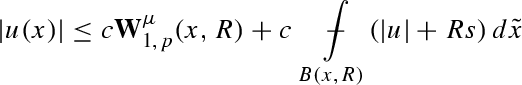(20)

holds whenever $B ( x , R ) ⊂ Ω$ and the right hand side is finite. Moreover, if

$lim R → 0 W 1 , p μ ( x , R ) = 0 locally uniformly in Ω w.r.t. x$
(21)

then $u$ is continuous in $Ω$.

A sketchy proof of this theorem is proposed in Sect. 18 below; extensions to more general operators have been given in [50, 53]. If $p = 2$, then $W 1 , p μ ≡ I 2 μ$ and we retrieve a local analog of the first estimate in (5). A remarkable point here is that estimate (20) is sharp, and the nonlinear potential $W 1 , p μ$ cannot be replaced by any other smaller potential. This is in fact reported in the following:

### Theorem 2

[37, 38] Let $u ∈ W 1 , p ( Ω )$ be a nonnegative weak solution to the Eq. (13) under the assumptions (18), where $μ$ is a positive measure and $s = 0$. There exists a constant $c ≡ c ( n , p , ν , L )$ such that the following pointwise estimate holds whenever $B ( x , 2 R ) ⊂ Ω$ and the Wolff potential is finite:

$c - 1 W 1 , p μ ( x , R ) ≤ u ( x ) ≤ c W 1 , p μ ( x , 2 R ) + c inf B ( x , R ) u .$

The possibility of extending pointwise potential estimates to the gradient of solutions has remained an open and discussed issue since the paper . The answer came only recently and here we consider the case of equations of the type (13). The first result in this direction is contained in  for the case $p = 2$, that is when assumptions (14) are in force. In  it is indeed proved the following analog of the second estimate in (5):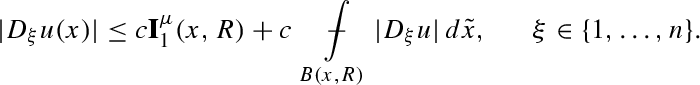(22)

For the case $p > 2$ a first result has been given in , where the following, apparently natural estimate, has been proved to hold at every Lebesgue point $x$ of $D u$, under assumptions (16):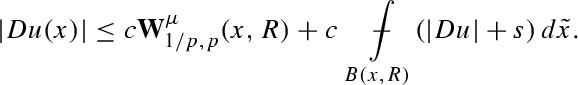(23)

The previous estimate seems to put a final word on the problem of a sharp analog of the second estimate in (5) since the orthodoxy of nonlinear potential theory prescribes that Wolff potentials replace Riesz potentials everywhere when $p ≠ 2$. Surprisingly enough, in  we have shown that this is not the case. Using Wolff potentials is necessary only when estimating solutions, while it is not when passing to their gradients. In fact the following holds:

### Theorem 3

 Let $u ∈ W 1 , p ( Ω )$ be a solution to the Eq. (13), under the assumptions (16). There exists a constant $c ≡ c ( n , p , ν , L )$ such that the Riesz potential estimate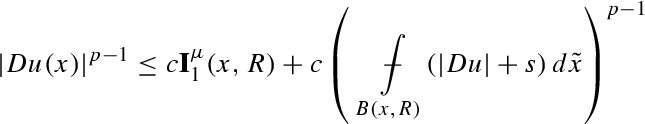(24)

holds whenever $B ( x , R ) ⊂ Ω$ and the right hand side is finite. Moreover, if

$lim R → 0 I 1 μ ( x , R ) = 0 locally uniformly in Ω w.r.t. x$
(25)

then $D u$ is continuous in $Ω$.

The proof of Theorem 3 will be presented in Sect. 15 below, where it will be obtained as a corollary of more general potential estimates. An extension of the previous result to a class of general operators including the $p$-Laplacean has been recently given by Baroni in .

### Remark 1

An obvious ambiguity arises in the statements of Theorems 1–3 when saying that the related estimates hold whenever the right hand side is finite. This might appear obvious. Another point is that estimates in Theorems 1–3 are stated for every $x$, while both $u ( x )$ and $D u ( x )$ are only defined if $x$ is Lebesgue point, where the so called precise representative can be defined. Both ambiguities are clarified in Sect. 8. There we prove that, both for $u$ and $D u$, the set of Lebesgue points coincides with the one for which inequalities (20) and (24) feature a finite right hand side, respectively.

Observe that estimate (24) obviously improves the one in (23) as, if $p ≥ 2$ then $1 / ( p - 1 ) ≤ 1$, and therefore

$I 1 μ ( x , R ) = ∫ 0 R | μ | ( B ( x 0 , ϱ ) ) ϱ n - 1 d ϱ ϱ ≲ ∑ i = 0 ∞ | μ | ( B ( x , R / 2 i ) ) ( R / 2 i ) n - 1 ≲ ∑ i = 0 ∞ | μ | ( B ( x , R / 2 i ) ) ( R / 2 i ) n - 1 1 / ( p - 1 ) p - 1 ≲ ∫ 0 2 R | μ | ( B ( x 0 , ϱ ) ) ϱ n - 1 1 / ( p - 1 ) d ϱ ϱ p - 1 = W 1 / p , p μ ( x , 2 R ) p - 1 .$
(26)

The implications of Theorem 3 are rather surprising: when switching to the gradient, estimates linearize and they reduce to the ones already available for the Poisson equation. The nonlinear, possibly degenerate character of the equations considered plays no role here. In particular, the regularity theory of equations as (13) reduces to that of the Poisson equation up to the $C 1$-level. Several facts typical of the linear theory can now be reproduced verbatim. For instance, for solutions $u ∈ W 1 , p ( R n )$ to (13) defined in the whole $R n$, the classical estimate via classical Riesz potentials

$| D u ( x ) | p - 1 ≤ c ∫ R n d | μ | ( y ) | x - y | n - 1$

follows immediately. For a relevant connection between Theorem 3 and fundamental solutions to general nonlinear measure data problems we refer to Sect. 7.1 below. Another very precise analogy with the classical linear theory is a striking nonlinear extension of a fundamental theorem of Stein . This claims that if $v ∈ W 1 , 1$ is a Sobolev function defined in $R n$ with $n ≥ 2$, then

$D v ∈ L ( n , 1 ) ⟹ v is continuous .$
(27)

This can be regarded as the limiting case of Sobolev–Morrey embedding theorem that reads $D v ∈ L n + ε ⟹ v ∈ C 0 , ε / ( n + ε )$ whenever $ε > 0$. Note indeed that $L n + ε ⊂ L ( n , 1 ) ⊂ L n$ for every $ε > 0$ with all the inclusions being strict. Another way to state Stein’s theorem concerns the regularity of solutions $u : Ω → R N$ to the Laplacean system, and reads

$div D u = ▵ u ∈ L ( n , 1 ) ⟹ D u is continuous .$

This follows by (27) and classical Calderón–Zygmund theory. The point is now that if $μ ∈ L ( n , 1 )$, then a computation involving the definition of norm in Lorentz spaces (see [23, 41] for discussion) implies that (25) takes place, and therefore we conclude with the following:

### Theorem 4

(Nonlinear Stein theorem ) Let $u ∈ W 1 , p ( Ω )$ be a solution to the Eq. (13), under the assumptions (16) and such that $μ ∈ L ( n , 1 )$ locally in $Ω$. Then $D u$ is continuous in $Ω$.

See  for a global Lipschitz bound. Without appealing to potentials, but by using different means, the result of the previous theorem also holds for systems.

### Theorem 5

(Vectorial nonlinear Stein theorem ) Let $u ∈ W 1 , p ( Ω , R N )$, $N ≥ 1$, be a vector valued solution to the $p$-Laplacean system

$- ▵ p u = F .$

Assume that the components of the vector field $F : Ω → R N$ locally belong to the space $L ( n , 1 )$. Then $D u$ is continuous in $Ω$.

Finally, let us mention that starting from the techniques developed for the last two theorems, similar results can be proved in the case of fully nonlinear equations .

## Universal potential estimates

The results of this section have a double aim. On the one hand, they show that estimates (9)–(10) have analogs for nonlinear equations. On the other hand, they show that those in (20), (23) and (24) are actually special cases of a more general class of nonlinear potentials estimates. These, in turn, allow to recover in an optimal way all the basic regularity properties of solutions in terms of the regularity of the assigned datum $μ$. The range of the results implied by such estimates is of course limited by the regularity theory of homogeneous equations as

$div a ( x , D w ) = 0 .$
(28)

Therefore we first recall what is the maximal regularity of solutions to equations as in (28) and in (29) below. In turn, this dramatically changes according to the smoothness assumed on the partial map $x → a ( x , · )$. When measurability is considered, very low degree of regularity is expected and solutions are just Hölder continuous for some exponent. When instead dependence on $x$ becomes more regular then higher regularity follows. In this case, again for sake of simplicity, and since this does not affect the expositions of the main ideas, we shall confine ourselves to the case of equations with no coefficients as

$div a ( D w ) = 0 .$
(29)

The first result we present upgrades estimate (20) to low order fractional derivatives, and allows to give a sharp formulation of the classical De Giorgi’s theory via nonlinear Wolff potential estimates. De Giorgi’s theory for equations with measurable provides the existence of a universal Hölder continuity exponent$α m ∈ ( 0 , 1 )$, depending only on $n , p , ν , L$, such that

$w ∈ C loc 0 , α ( Ω ) for every α < α m$
(30)

and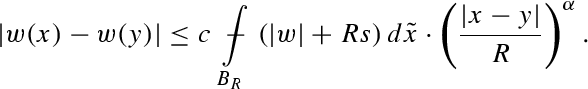(31)

The previous estimate holds whenever $x , y ∈ B R / 2$ and $B R ⊂ Ω$, for a constant depending only on $n , p , ν , L$ and $α$. The exponent $α m$ can be thought as the maximal Hölder regularity exponent associated to the vector field $a ( · )$. It is universal in the sense that it is independent of $a ( · )$ and of the particular solution considered, but just depends only on $n , p , ν , L$. For more precise information on $α m$ see Theorem 18 and Remark 6 below. It then holds the following:

### Theorem 6

(De Giorgi’s theory via potentials ) Let $u ∈ W 1 , p ( Ω )$ be a weak solution to the equation with measurable coefficients (17) under assumptions (18). Let $B R ⊂ Ω$ be such that $x , y ∈ B R / 2$; then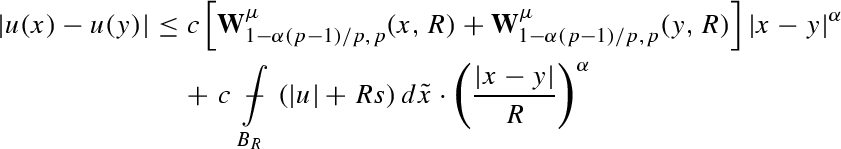(32)

holds provided the right hand side is finite and $0 ≤ α < α m$, where the exponent $α m$ has been defined in (30)–(31). Moreover, whenever $α ~ ∈ [ 0 , α m )$ is fixed, the dependence of the constant $c$ is uniform for $α ∈ [ 0 , α ~ ]$, in the sense that $c$ depends only on $n , p , ν , L$ and $α ~$.

Notice that when $μ ≡ 0$ estimates (31) and (33) coincide. In general, counterexamples show that in (30) $α m → 0$ when $L / ν → ∞$. This prevents estimate (33) to hold in general for the full range $α ∈ [ 0 , 1 )$ when $x → a ( x , · )$ is measurable. Things change when the dependence on $x$ is more regular. For instance, when considering Eq. (29), estimate (31) holds for $α = 1$. In this case the validity of (32) extends to the whole interval $α ∈ [ 0 , 1 ]$. Moreover, estimates (20) and (23) can be uniformly obtained as limiting endpoint cases of (32) for $α = 0$ and $α = 1$, respectively. In fact, it holds the following:

### Theorem 7

(Uniform Wolff potential estimate ) Let $u ∈ W 1 , p ( Ω )$ be a weak solution to the Eq. (13) under assumptions (16). Let $B R ⊂ Ω$ be such that $x , y ∈ B R / 2$; then estimate (32) holds for $α ∈ [ 0 , 1 ]$, whenever the right hand side is finite. The constant $c$ depends only on $n , p , ν , L$ but is otherwise independent of $α$.

Estimate (32) does not catch up, when $α → 1$, the optimal gradient bound in (24). There is a natural reason for such a lack of endpoint property: it catches the other optimal bound (20) as $α → 0$, and the two cases involve different potentials. In fact, the change in the nature of the estimates when passing from (20) to (24) requires another theorem, parallel to Theorem 7.

### Theorem 8

(Uniform Riesz potential estimate) Let $u ∈ W 1 , p ( Ω )$ be a weak solution to the Eq. (13) under assumptions (16). Let $B R ⊂ Ω$ be such that $x , y ∈ B R / 4$; then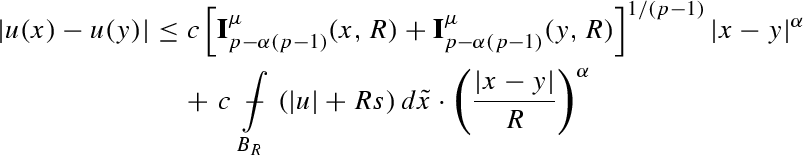(33)

holds provided the right hand side is finite and $0 < α ≤ 1$. Moreover, whenever $α ~ ∈ ( 0 , 1 ]$ is fixed, the dependence of the constant $c$ is uniform for $α ∈ [ α ~ , 1 ]$ as $c$ depends only $n , p , ν , L$ and $α ~$.

The previous result is here presented for the first time and for the proof we refer to Sect. 5 below. There Theorem 8 is actually obtained as a corollary of a more general estimate for certain maximal operators of $D u$. From the discussion made there it will be clear that the points $x , y$ are Lebesgue points of $u$ when the right hand side in (33) is finite; compare with Remark 1. Theorems 6 and 8 provide altogether the optimal analog of estimate (9) and in fact they both unify with Theorem 7 when $p = 2$, when Wolff and Riesz potentials do coincide. We remark that is also possible to quantify the blow-up of the constant $c$ in (33) as $α → 0$; see Remark 7 below.

We now examine the situation for the gradient, giving an estimate which is again bound to give back (10) when $p = 2$. As already done before Theorem 6, we recall the basic information about the maximal regularity of solutions to homogeneous equations as in (29). These in turn involve the fundamentals of the regularity theory of the $p$-Laplacean equations and can be summarised by saying that there exists a positive exponent $α M ∈ ( 0 , 1 )$, depending only on $n , p , ν$ and $L$, such that whenever $x , y ∈ B R / 2$,

$D w ∈ C loc 0 , α ( Ω ) for every α < α M$
(34)

and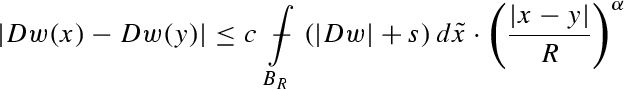(35)

hold for any local solution$w$ to (29). The constant $c$ depends only on $n , p , ν , L$ and $α$. For estimate (35) and the exponent $α M$ see Theorem 17 and Remark 5 below. The result in (34) finds its origins in the basic work of Uraltseva  while further approaches can be found in [18, 24, 46, 51]. We remark that all these results are again based on the groundbreaking work of De Giorgi . The exponent $α M$ is in general strictly less than one (see again ); while lower bounds on $α M$ can be obtained by tracking the constant dependence in the various proofs, its precise (optimal) value is not known. An optimal lower bound for $α M$ is conjectured to be $1 / 3$, according to the regularity exhibited by the solutions to the so called $∞$-Laplacean equation . By taking (34) into account we have the following nonlinear version of estimate (10):

### Theorem 9

(Uniform Wolff potential estimate) Let $u ∈ W 1 , p ( Ω )$ be a weak solution to the Eq. (13) under assumptions (16). Let $B R ⊂ Ω$ be such that $x , y ∈ B R / 4$; then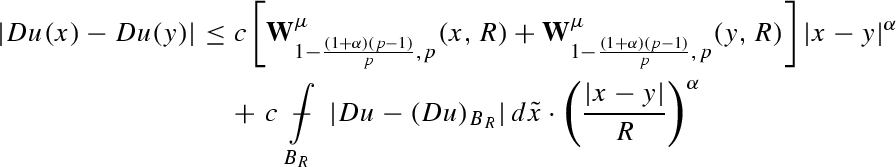(36)

holds provided the right hand side is finite and

$0 ≤ α < min { 1 / ( p - 1 ) , α M } .$
(37)

The exponent $α M$ has been defined in (34). Moreover, whenever $0 ≤ α ~ < min { 1 / ( p - 1 ) , α M }$ is fixed, the dependence of the constant $c$ is uniform for $α ∈ [ 0 , α ~ ]$, in the sense that $c$ depends only $n , p , ν , L$ and $α ~$.

The proof of this theorem will be presented in Sect. 16 below. Notice that the limitation in (37) makes the statement of Theorem 9 consistent with the definition of Wolff potential given in Definition 5, where it must be $β > 0$.

## Maximal-potential estimates

Let us now explain Theorem 8 proceeding for some while with a few purely heuristic arguments. By considering the function $m$ appearing in (11) as a fractional derivative of order $α$, we can express the content of Theorem 8 in the following way:

$| ∂ α u ( x ) | p - 1 ≲ I p - α ( p - 1 ) ( | μ | ) ( x ) , 0 < α ≤ 1$
(38)

thereby obtaining a nonlinear version of (12). The singularity of the case $α = 0$ essentially stems from the fact that Wolff potentials come then into the play when considering pointwise estimates for $u$. Let us proceed heuristically and see why Riesz potentials intervene as long as fractional derivatives are considered. We rewrite Eq. (15) as the decoupled system

$- div H = μ H = | D u | p - 2 D u .$

This viewpoint tells us that an equation as in (15), which is commonly seen as a nonlinear equation in the gradient, can be also seen as linear equation in a nonlinear vector field of the gradient, that is $H$. The analysis of equations as $- div H = μ$, being $f → div f$ a differential operator of order one, typically involves estimates of $H$ via operators as $I 1 μ$. In turn this explains the appearance of estimates as in (24). The reader will immediately recognize that no similar argument applies to $u$, as the equation depends directly on $D u$ and not $u$. This is ultimately the reason for the appearance of Wolff potentials when deriving pointwise for $u$ as (20). Let us now go to intermediate derivatives and let us believe that fractional derivatives $∂ α u$ can be controlled by in turn controlling quantities as

$| u - ( u ) B R | R α ≈ R 1 - α | D u | ≈ | ∂ α u |$
(39)

on every possible ball $B R$. Using a typical dimension analysis viewpoint, the appearance in the estimates of a multiplicative factor as $R - β$ amounts to consider a corresponding derivative of order $β$ in the estimates. Now, let us rewrite Eq. (15) as

$R ( α - 1 ) ( p - 1 ) div ( | R 1 - α D u | p - 2 R 1 - α D u ) = μ$

and note that in this scheme of reasoning the operator $f → R ( α - 1 ) ( p - 1 ) div f$ has now order $p - α ( p - 1 )$. Its inversion should therefore involve potentials as $I p - α ( p - 1 ) μ$. At this point, recalling the identification (39) leads to conclude with an estimate with the dimensional meaning of (38). The point is now to make everything rigorous. A natural way to make the identification in (39) is to use a nonlocal operator as for instance a fractional maximal operator. It is therefore time for the following:

### Definition 6

(Fractional maximal operator) Let $β ∈ [ 0 , n ]$, $x ∈ Ω$ and $R < dist ( x , ∂ Ω )$, and let $f$ be an $L 1 ( Ω )$-function or a measure with finite mass; the function defined by

$M β , R ( f ) ( x ) : = sup 0 < r ≤ R r β | f | ( B ( x , r ) ) | B ( x , r ) |$

is called the restricted (centered) fractional $β$ maximal function of $f$.

Using fractional maximal operators allows to get Riesz potential estimates which are uniform in the whole range $[ 0 , 1 ]$, and that provide a rigorous interpretation of previous heuristic arguments. The outcome is summarized in the following theorem, which appears here for the first time. The proof is contained in Sect. 12 below (see Sect. 14.4 for the case of SOLA).

### Theorem 10

(Uniform maximal-potential estimates) Let $u ∈ W 1 , p ( Ω )$ be a weak solution to the Eq. (13) under assumptions (16). Then for every ball $B ( x , R ) ⊂ Ω$ the following estimate: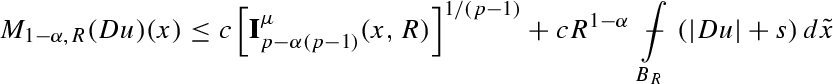(40)

holds uniformly in $α ∈ [ 0 , 1 ]$, with $c ≡ c ( n , p , ν , L )$.

The maximal operators of Definition 3 naturally connect to those presented in Definition 6 via Poincaré inequality

$M α , R # ( u ) ( x ) ≤ c M 1 - α , R ( D u ) ( x ) , α ∈ [ 0 , 1 ]$
(41)

so that a uniform estimate on $M α , R # ( u )$ follows appealing to Theorem 10, that is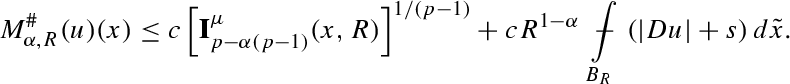(42)

We yet remark that in the estimate in the last display the constant $c$ is independent of $α$, and in fact the estimate holds uniformly for $α ∈ [ 0 , 1 ]$.

The turning point to pointwise estimates is now given by the fact that the sharp maximal operator $M α , R # ( u ) ( x )$ controls the pointwise behaviour of $u$ provided $α > 0$. This fact is expressed in the following Proposition, a first form of which is present in . It in turn relies on some original arguments of Campanato .

### Proposition 1

Let $f ∈ L 1 ( B 8 R / 5 )$; for every $α ∈ ( 0 , 1 ]$ the inequality

$| f ( x ) - f ( y ) | ≤ c α M α , R # ( f ) ( x ) + M α , R # ( f ) ( y ) | x - y | α$
(43)

holds whenever $x , y ∈ B 2 R / 5$, for a constant $c$ depending only on $n$. More precisely, $x$ and $y$ are Lebesgue points of $f$ whenever $M α , R # ( f ) ( x )$ and $M α , R # ( f ) ( y )$ are finite, respectively. Therefore, whenever the right hand side in (43) is finite, the values of $f$ are defined as follows:

$f ( x ) : = lim ϱ → 0 ( f ) B ( x , ϱ ) and f ( y ) : = lim ϱ → 0 ( f ) B ( y , ϱ ) .$

Proposition 1 allows to obtain Theorem 8 as a corollary of Theorem 10 and in particular of (42); see Sect. 12.7 below for the proofs, including the one of Proposition 1. We believe that Theorem 10 helps to understand the peculiar nature of the case $α = 0$ and the occurrence of Wolff potentials instead of Riesz potentials. Indeed, an $L ∞$-bound on the sharp maximal operator is essentially equivalent to require that $u$ belongs to the space BMO (introduced in , see (68) below). On the other hand, a real $L ∞$-bound on $u$ itself requires an $L ∞$-bound on $W 1 , p μ$. This situation looks completely natural when considering typical examples of functions lying in BMO$\ L ∞$, as for instance $log | x |$. The $log$-scale is indeed the one making a difference between a potentials as $W 1 , p μ$ and $I 1 μ$. Taking for instance

$μ ( x ) = 1 | x | p log β | x |$

we see that $I p μ ( 0 , 1 ) < ∞$ provided $β > 1$ while $W 1 , p μ ( 0 , 1 ) < ∞$ provided $β > p - 1$. In the next section we shall in fact describe a few additional results showing that also the case $α = 1$ reveals to be special when using maximal operators instead of potentials.

### Non-endpoint maximal estimates

An interesting fact concerning the two cases $α = 0 , 1$ of (40) is that when looking for bounds on intermediate derivatives, potentials can be replaced by maximal operators. These give in fact smaller quantities. Maximal operators, as we shall see, allow to replace conditions typically given in Lebesgue or Lorentz spaces, with weaker conditions formulated in terms of Marcinkiewicz spaces. Let us recall the following, elementary inequality which holds for $γ ∈ ( 0 , 1 )$

$M β , γ R ( μ ) ( x ) ≤ c ( n , γ , β ) I β μ ( x , R ) ,$

see for instance [40, Lemma 4.1]. Then we have the following:

### Theorem 11

(Intermediate maximal estimates ) Let $u ∈ W 1 , p ( Ω )$ be a weak solution to (13) under the assumptions (16). Let $B R ⊂ Ω$ be a ball centred at $x$; then the estimate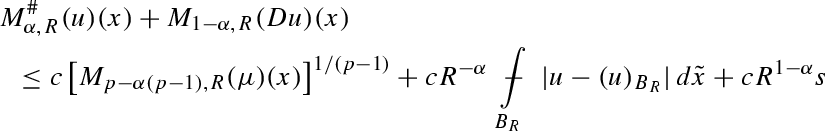(44)

holds uniformly in $α ∈ [ 0 , α ~ ]$, whenever $0 < α ~ < 1$. The constant $c$ depends only on $n , p , ν , L , α ~$.

As for gradient oscillations, we instead have the following result, whose proof is contained in Sect. 13 below.

### Theorem 12

(Gradient sharp maximal estimate ) Let $u ∈ W 1 , p ( Ω )$ be a weak solution to (13) under the assumptions (16). Let $B R ⊂ Ω$ be a ball centered at $x$; then the following estimate: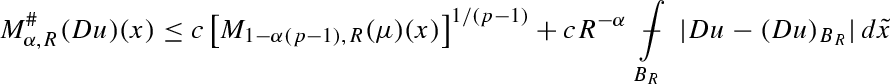holds uniformly in $α ∈ [ 0 , α ~ ]$ whenever

$0 ≤ α ~ < min { 1 / ( p - 1 ) , α M } ,$

for a constant $c$ depending only on $n , p , ν , L , α ~$. The exponent $α M$ has been introduced in (34)–(35).

## Equations with coefficients

Getting nonlinear potential estimates catching regularity beyond Theorem 6 for equations as in (17), necessitates to assume more regularity on the partial map $x ↦ a ( x , · )$. Keep in mind the discussion before Theorem 6. We therefore define the following averaged vector field: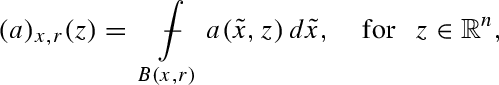(45)

whenever $B ( x , r ) ⊂ Ω$, and then the averaged (and renormalized) modulus of continuity of $x ↦ a ( x , · )$ as follows: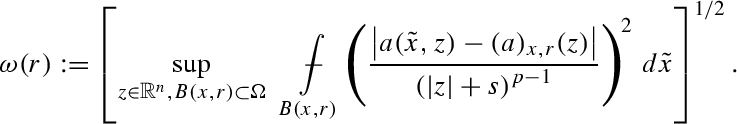(46)

When considering equations of the type

$- div ( c ( x ) a ~ ( D u ) ) = μ$
(47)

the definition in (46) gives something which is comparable to the usual modulus of continuity of the function $c ( · )$. We then have

### Theorem 13

Let $u ∈ W 1 , p ( Ω )$ be a weak solution to (17), with assumptions (16) (uniformly) verified by the partial map $z → a ( · , z )$ for every $x ∈ Ω$. Then

•  For every $α ~ ∈ ( 0 , 1 )$ there exists a number $δ ∈ ( 0 , 1 )$, depending only on $n , p , ν , L , α ~$, such that

$lim sup ϱ → 0 ω ( ϱ ) ≤ δ$
(48)

implies the validity of estimate (32) whenever $α ∈ [ 0 , α m )$. In particular, if the limit in (48) is zero estimate (32) holds for every $α < 1$

•  If the partial map $x → a ( x , · )$ is Dini-continuous, i.e.

$∫ 0 ω ( ϱ ) d ϱ ϱ < ∞ ,$
(49)

then estimates (33) and (40) continue to hold, for $α ∈ [ α ~ , 1 ]$ and $α ∈ [ 0 , 1 ]$, respectively

•  If the partial map $x → a ( x , · )$ is Hölder continuous with exponent $α ~ < min { 1 / ( p - 1 ) , α M }$ in the sense that

$sup r ∫ 0 r [ ω ( ϱ ) ] 2 / p ϱ α ~ d ϱ ϱ < ∞ ,$
(50)

then estimate (36) holds whenever $α < α ~$.

In all three cases the constants involved in the estimates additionally depend on the quantities appearing in (48)–(50).

Let us now briefly comment on the assumptions made in the last theorem, remarking that all of them are essentially necessary. Assumption (48) allows to catch the case in which, when referring to Eq. (47), the function $c ( · )$ is not even continuous, but of class VMO or BMO with small seminorm. This reconnects the theory presented here to the classical Calderón–Zygmund theory, where VMO-regularity of coefficients is required to establish integrability estimates related to estimate (32); see for instance . Assumption (149) is necessary too. Indeed, estimate (33) implies gradient boundedness when $μ$ is good enough, while Dini continuity of coefficients is known to be necessary to get Lipschitz regularity of solutions; see . The proof of the second statement in the last theorem has the techniques developed for Theorem 10 as starting point, and will appear in the forthcoming paper . Finally, assumption (50) seems to be natural in view of the usual Schauder theory. This prescribes that, in order to have Hölder continuity of the gradient, the Hölder continuity of $x ↦ a ( x , · )$ is necessary.

## Interlude on measure data problems

When looking at equations as in (17) we have considered distributional solutions lying in $W 1 , p$. This choice is actually aimed at simplifying the presentation in a way that emphasises the results obtained in the form of a priori estimates. Dealing with $W 1 , p$-solutions is anyway very much restrictive in view of the fact that, typically, distributional solutions to measure data problems do not enjoy this regularity. A typical instance is the so called nonlinear Green’s function

$G p ( x ) ≈ | x | p - n p - 1 - 1 if 1 < p ≠ n log | x | if p = n$
(51)

which solves, in the sense of distributions, the problem

$- ▵ p u = δ in B ( 0 , 1 ) u = 0 on ∂ B ( 0 , 1 ) .$
(52)

In the right hand side of (52)$1$ there appears the Dirac measure $δ$ charging the origin. A straightforward calculation reveals that $G p ∉ W 1 , p$ while it holds that

$| D G p | p - 1 ∈ M n / ( n - 1 ) ⟺ | { | D G p | p - 1 > λ } | ≲ λ - n / ( n - 1 ) .$
(53)

We recall here that the Marcinkiewicz space $M q ( Ω )$, for $1 ≤ q < ∞$, is the set of all measurable maps $μ$ satisfying the condition

$sup λ ≥ 0 λ q | { x : | μ | ( x ) > λ } | < ∞ .$
(54)

The above discussion now motivates the following:

### Definition 7

(Very weak solutions) A function $u ∈ W loc 1 , 1 ( Ω )$ is called a very weak solution $u$ to the Eq. (17) in $Ω$ if $a ( x , D u ) ∈ L loc 1 ( Ω , R n )$ and (19) holds for every $φ ∈ C c ∞ ( Ω )$.

The terminology “very weak” is used to emphasize the fact that such solutions do not belong, in general, to the natural Sobolev space $W 1 , p ( Ω )$, i.e. they are not energy solutions. Note that, when considering assumptions (18), in order to guarantee that $a ( x , D u ) ∈ L 1 ( Ω , R n )$ it suffices, for instance, to have $D u ∈ W 1 , p - 1 ( Ω )$. Definition 7, not surprisingly, poses problems. For instance, very weak solutions may exist beside usual energy solutions , and are therefore not in general unique. As a matter of fact one of the basic open issues of the theory of measure data problems is to find a function class where to solve in a unique way Dirichlet problems of the type

$- div a ( x , D u ) = μ in Ω u = 0 on ∂ Ω ,$
(55)

with $μ$ being in the most general case a (signed) Borel measure with finite total mass. We will not pursue this matter here, rather referring to [14, 36] for a more comprehensive discussion. Here we are interested in a class of solutions which we regard to be very natural (see in fact the equivalence results obtained in ) and for which all the potential estimates described in this paper continue to hold. This is the class of SOLA (Solutions Obtained by Limits of Approximations), which is introduced in the

### Definition 8

(SOLA) A function $u ∈ W loc 1 , 1 ( Ω )$ is a SOLA to the Eq. (17) iff is a very weak solution and there exists a sequence of local energy solutions ${ u k } ⊂ W loc 1 , p ( Ω )$ to the equations

$- div a ( x , D u k ) = μ k ,$

such that $u k → u$ locally in $W 1 , p - 1 ( Ω )$.

SOLA are special because they are selected via an approximation procedure using more regular energy solutions, and thereby they inherit a few of their basic properties. For instance: their precise representative is defined out of a null $p$-capacity set, exactly as $W 1 , p$-functions; see Theorem 16 below. Let us recall how to build a SOLA to (55), following . One considers solutions $u k ∈ W 0 1 , p ( Ω )$ to regularised problems

$- div a ( x , D u k ) = μ k in Ω u k = 0 on ∂ Ω .$

The right hand sides data $μ k : = μ ∗ ϕ k ∈ C ∞$ are canonically obtained by smoothing $μ$ via convolution with a sequence of smooth standard, smooth mollifiers ${ ϕ k }$. This approach leads to the existence of a very weak solution $u ∈ W 0 1 , p - 1 ( Ω )$ such that $u k → u$ in $W 0 1 , p - 1 ( Ω )$ (up to a not relabeled subsequence). SOLA are not known to be unique, except in a few special cases (for instance when $μ ∈ L 1$). See [8, 13, 14, 36] for a larger discussion. We summarise the basic existence and regularity results available for SOLA in the following:

### Theorem 14

[8, 55] Under the assumptions (18) with $p ≤ n$, there exists a SOLA $u ∈ W 0 1 , p - 1 ( Ω )$ to (55). Moreover, every SOLA $u ∈ W loc 1 , 1 ( Ω )$ to (17) is such that

$u ∈ W loc 1 , q ( Ω ) for every q < n ( p - 1 ) / ( n - 1 ) .$
(56)

In the case of equations of the type (13) under assumptions (16), we also have

$D u ∈ W loc ( 1 - ε ) / ( p - 1 ) , p - 1 ( Ω ) for every ε ∈ ( 0 , 1 ) .$
(57)

A preliminary integrability result was obtained for a different kind of solutions in the pioneering paper of Lindqvist . The restriction $p ≤ n$ is aimed at focusing on the case of SOLA, since when $p > n$ then $μ$ belongs to the dual of $W 1 , p$ and it is possible to consider standard energy solutions. In display (57) a fractional Sobolev space appears. The meaning is actually that

$∫ A ∫ A | D u ( x ) - D u ( y ) | p - 1 | x - y | n + 1 - ε d x d y < ∞ for every ε ∈ ( 0 , 1 )$

holds whenever $A ⋐ Ω$. We also remark that, in fact, it can be proved that every SOLA $u$ is such that

$| D u | p - 1 ∈ M n / ( n - 1 ) ( Ω ) .$
(58)

This means that every SOLA exhibits exactly the same integrability of the nonlinear Green’s function $G p$ described in (53) (see [7, 20, 55]). Both the results in (56) and (57) are sharp, as follows again considering $G p$, which can be in fact proved to be the only SOLA to the problem in (52). This last fact follows combining the results in [35, 62]. As mentioned above, by mean of approximations arguments, all the potential estimate in this paper continue to hold for any SOLA to (55). More details are in Sect. 14.4 below, where we in particular discuss the possibility of characterizing the Lebesgue points of SOLA via linear and nonlinear potentials.

### Potential estimates and fundamental solutions

To check to which extent estimates (20) and (24) replace the usual linear representation formulas in the nonlinear case, the best thing to do is to see how they reproduce the behaviour of the nonlinear fundamental solution $G p$. We already know the optimality of estimate (20) from Theorem 2. We therefore concentrate on (24) and show that it actually reverses when considering the nonlinear Green’s function $G p$. This means that for $x ∈ B ( 0 , 1 ) \ { 0 }$ we have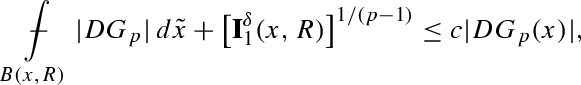(59)

with $R = 2 | x |$, and for a constant $c$ depending only on $n$ and $p$. Indeed by coarea formula we have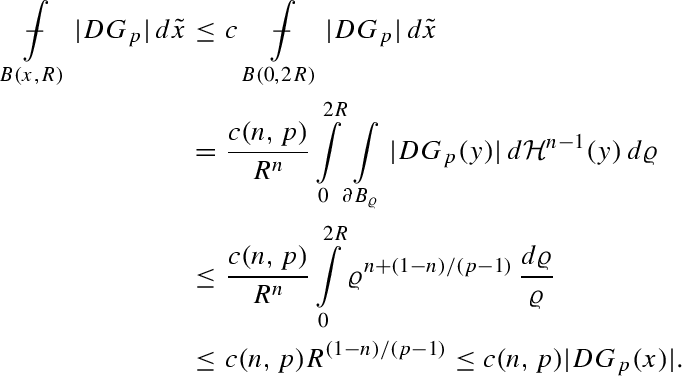On the other hand, as $δ ( B ( x , ϱ ) ) = 0$ for $ϱ ≤ R / 2$ and $δ ( B ( x , ϱ ) ) = 1$ otherwise, we also have

$I 1 δ ( x , R ) = ∫ R / 2 R d ϱ ϱ n = 2 n - 1 n - 1 R 1 - n ≤ c ( n , p ) | D G p ( x ) | p - 1 ,$

so that (59) follows combining the last two inequalities.

## Fine properties of solutions via potentials

We have seen that linear and nonlinear potentials locally control the behaviour of solutions. It is at this point not surprising to discover that potentials also control their so called fine properties. In this respect we present two theorems. The former is concerned with the pointwise behaviour of gradients of SOLA, and in particular with their Lebesgue points. This result employs Riesz potentials. The latter in instead concerned with Lebesgue points of solutions and uses Wolff potentials.

### Theorem 15

Theorem 3 continues to hold whenever $u ∈ W 1 , p - 1 ( Ω )$ is a SOLA to (13). Moreover, the condition

$I 1 μ ( x , R ) < ∞$
(60)

implies that the following limit exists and therefore defines the precise representative of $D u$ at the point $x$:

$lim ϱ → 0 ( D u ) B ( x , ϱ ) = : D u ( x ) .$
(61)

The proof of this last theorem is included in Sect. 17 below. The analogous statement for solutions is the following:

### Theorem 16

Theorem 1 continues to hold whenever $u ∈ W 1 , p - 1 ( Ω )$ is a SOLA to (17). Moreover, the condition

$W 1 , p μ ( x , R ) < ∞$
(62)

implies that the following limit exists and therefore defines the precise representative of $u$ at the point $x$:

$lim ϱ → 0 ( u ) B ( x , ϱ ) = : u ( x ) .$
(63)

In particular, the set of non-Lebesgue points of $u$ has $p$-capacity zero.

We notice that the assertion about the $p$-capacity follows directly from the fact that set of points where the Wolff potential $W 1 , p μ$ blows-up has zero $p$-capacity (see for instance ).

### Remark 2

(Hausdorff dimension of singular sets of SOLA) Theorem 16 allows to define SOLA—which are initially defined only almost everywhere via convergence—outside a singular set (i.e. set of non-Lebesgue points) of Hausdorff dimension not larger than $n - p$, when $p ≤ n$. This establishes a connection with another class of solutions to measure data problem. These are called $p$-superharmonic functions and are defined in the case the measure $μ$ is nonnegative; see [30, 38]. For such solutions every point is a Lebesgue point, by construction. The connection is now given by the fact that, in view of , every SOLA has a superharmonic representative (that is, they coincide almost everywhere) whenever the measure is nonnegative. In the case of Theorem 15 we instead can conclude that the Hausdorff dimension of the singular set of $D u$ is not larger than $n - 1$. The last estimate follows on the other hand also by (57). Indeed, the Hausdorff dimension of the set of non-Lebesgue points of a general $W s , γ$-map, with $s γ < n$, has Hausdorff dimension not larger that $n - s γ$; see .

### Remark 3

(Analogies with linear potential theory) Theorems 15 and 16 can be also considered as analogs of classical facts in linear potential theory, concerning the pointwise behaviour of solutions to the Poisson equation $- ▵ u = μ$. As usual, in the linear case they follow from explicit representation formulas and abstract analysis of potentials. Here they are replaced by nonlinear potential estimates. Indeed, define

$f ( x ) : = ( G α ∗ g ) ( x ) : = ∫ R n G α ( x - y ) g ( y ) d y ,$

where $g ∈ L q ( R n )$ with $q > 1$. Here $G α$ denotes the $α$-Bessel potential, that is defined via

$G α : = F - 1 ( 1 + | ξ | 2 ) - α / 2 ,$

where $F$ denotes the Fourier transform and $α ∈ ( 0 , n )$. Then the limit

$lim ϱ → 0 ( f ) B ( x , ϱ ) = : f ( x )$

exists—and thereby defines the precise representative of $f$—up to a set of zero $( α , q )$-capacity. For these facts we refer to , and in particular to [3, Proposition 6.1.3]

## Regularity and corollaries

The estimates presented up to now allow to give a comprehensive and unified picture of the regularity results available for quasilinear equations. We will now briefly describe a few consequences of such estimates. First of all, let us recall that combining Theorem 11 and Proposition 1 yields the following pointwise inequality: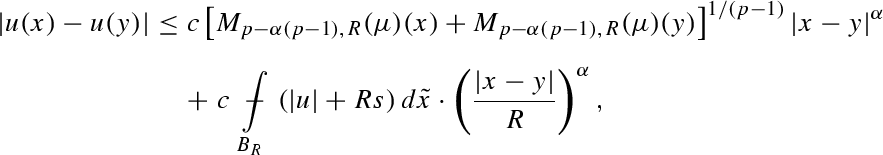(64)

that holds whenever $x , y ∈ B R / 4$, $B R ⊂ Ω$, $α ∈ ( 0 , 1 )$, where $c ≡ c ( n , p , ν , L , α )$. Notice here that the blow-up of $c$ takes place as soon as $α$ approaches $0$ or $1$. In a completely similar way, by Theorem 12 and again Proposition 1, we then have that the inequality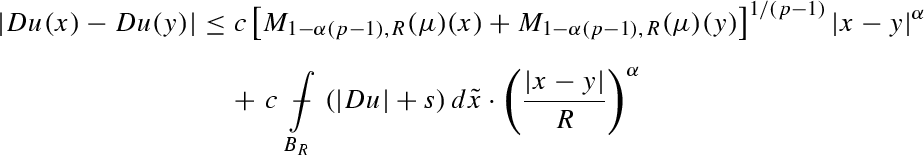(65)

holds with $x , y , r , B R$ and $c$ as above, but for $α ∈ ( 0 , α M )$. This time the constant $c$ blows-up when $α → 0$ or $α → α M$. We are now interested in seeing how the results presented up to now, including estimates (64)–(65), imply regularity of solutions in various relevant function spaces. Let us briefly recall that the Morrey space $L q , θ ( Ω )$ for $q ≥ 1$ and $0 ≤ θ ≤ n$, is defined by requiring that its member $f ∈ L q ( Ω )$ satisfies

$sup B ϱ ⊂ Ω ϱ θ - n ∫ B ϱ | f | q d x ~ < ∞ ,$
(66)

while their local version is defined in the usual way. We refer to [1, 56] for more on Morrey spaces and their basic properties with related references. We now have the following, comprehensive:

### Corollary 1

Let $u ∈ W 1 , p ( Ω )$ be a weak solution—or a SOLA—to the Eq. (13) under assumptions (16). Then, with all the spaces being meant in their local versions, we have that

• (C1) if $α ∈ ( 0 , 1 )$ and $q > n ( p - 1 ) / [ n - p + α ( p - 1 ) ]$, then

$μ ∈ L n q n ( p - 1 ) + [ p - α ( p - 1 ) ] q ⟹ u ∈ C q α$

(estimate (64))

• (C2) if $p < n$, then

$μ ∈ M n / p ⟹ | μ | ( B ϱ ) ≲ ϱ n - p ⟹ u ∈ BMO$

and

$p = n ⟹ u ∈ BMO$

(Theorem 11 with $α = 0 )$

• (C3) $W 1 , p μ ∈ L ∞ ⟹ u ∈ L ∞$ (Theorem 1)

• (C4) if $p < n$, then $μ ∈ L ( n / p , 1 / ( p - 1 ) ) ⟹ u ∈ C 0$ (Theorem 1)

• (C5) if $α ∈ ( 0 , 1 )$ and $p ≤ n$, then

$μ ∈ M n p - α ( p - 1 ) ⟹ | μ | ( B ϱ ) ≲ ϱ n - p + α ( p - 1 ) ⟹ u ∈ C 0 , α$

(estimate (64))

• (C6) if $p ≤ n$, then $μ ∈ M b ⟹ D u ∈ M n ( p - 1 ) / ( n - 1 )$ (Theorem 3)

• (C7) if $1 < q < n$, then

$μ ∈ L ( q , γ ) ⟹ D u ∈ L n q ( p - 1 ) n - q , γ ( p - 1 )$

(Theorem 3)

• (C8) if $1 < q < θ$, then $| D u | p - 1 ∈ L θ q / ( θ - q ) , θ$ (Theorem 3)

• (C9) $μ ∈ M n ⟹ | μ | ( B ϱ ) ≲ ϱ n - 1 ⟹ D u ∈$ BMO (Theorem 12)

• (C10) $I 1 μ ∈ L ∞ ⟹ D u ∈ L ∞$ (Theorem 8)

• (C11) $μ ∈ L ( n , 1 ) ⟹ D u ∈ C 0$ (Theorem 3)

• (C12) if $0 < α < min { 1 / ( p - 1 ) , α M }$, then

$μ ∈ M n 1 - α ( p - 1 ) ⟹ | μ | ( B ϱ ) ≲ ϱ n - 1 + α ( p - 1 ) ⟹ D u ∈ C 0 , α$

(estimate (65)).

### Proof

In order to prove (C1), we begin recalling the following mapping property of the fractional maximal operator:

$M β , R : L t → L n t / ( n - β t ) ,$
(67)

that holds whenever $1 < t < n / β$ (see [26, 56]). Then we go to estimate (64) and yet recall the definition of Calderón spaces given in Definition 2. This leads to determine

$n t n - [ p - α ( p - 1 ) ] t = q p - 1 ⟺ t = n q n ( p - 1 ) + [ p - α ( p - 1 ) ] q$

so that $μ ∈ L t$ and (67) with the above choice implies $[ M p - α ( p - 1 ) , R ( μ ) ] 1 / ( p - 1 ) ∈ L q$. At this point (C1) follows using this fact together with estimate (64). Notice that, recalling that we are assuming $p ≥ 2$, we have used that

$t = n q n ( p - 1 ) + [ p - α ( p - 1 ) ] q > 1 ⟺ q > n ( p - 1 ) n - p + α ( p - 1 ) > 1 .$

We have also use the fact with the previous definitions we in fact have that $t [ p - α ( p - 1 ) ] < n$.

The assertion in (C2) follows immediately by taking $α = 0$ in Theorem 11 when $p = n$. We indeed recall that by the very definition of BMO spaces we have

$M 0 , R # ( u ) ≡ M R # ( u ) ∈ L ∞ ⟺ u ∈ BMO .$
(68)

In the case $2 ≤ p < n$, we recall the following Hölder’s type inequality valid in Marcinkiewicz spaces $M t$, $t > 1$:

$‖ f ‖ L 1 ( B ϱ ) ≤ t ϱ n ( t - 1 ) / t t - 1 ‖ f ‖ M t ( B ϱ ) .$
(69)

This implies that in our case $| μ | ( B ϱ ) ≲ ϱ n - p$ holds and, by the definition of fractional maximal operator, we conclude that $M p , R ( μ )$ is locally bounded. At this stage (C2) follows from estimate (44), where we again take $α = 0$.

The statement in (C3) is simply an obvious consequence of Theorem 1.

As for (C4), a direct computation using certain characterisation of Lorentz spaces shows that if $μ ∈ L ( n / p , 1 / ( p - 1 ) )$ for $p < n$, then (21) holds (see for instance  for related computations). At this stage (C4) follows by Theorem 1.

In (C5) the first implication follows again by the Hölder type inequality in (69). On the other hand, we observe that the inequality $| μ | ( B ϱ ) ≲ ϱ n - p + α ( p - 1 )$ implies that $M p - α ( p - 1 ) , R ( μ ) ∈ L ∞$, locally, by the very definition of fractional maximal operator in Definition 6, so that (C5) follows by estimate (64).

(C6) is just an obvious consequence of the following imbedding property of Riesz potentials (see ):

$I 1 : M b → M n / ( n - 1 )$

and of Theorem 3.

Similarly, (C7) follows from Theorem 3 and the classical mapping property

$I 1 : L ( q , γ ) → L ( n q / ( n - q ) , γ ) for 1 < q < n and γ > 0 .$

(C8) is essentially a corollary of Theorem 3 and of the mapping property of Riesz potentials

$I 1 : L q , θ → L θ q / ( θ - q ) , θ ,$

The first implication in (C9) is a consequence of (69) while the second follows applying Theorem 12 with $α = 0$. Indeed, assuming that $| μ | ( B ϱ ) ≲ ϱ n - 1$ implies that $M 1 ( μ )$ is locally bounded, and therefore so is $M 0 , R # ( D u ) ≡ M R # ( D u )$. By definition of sharp maximal function this implies that the gradient belongs to BMO, locally; see (68).

(C10) follows from Theorem 8 in an obvious way.

(C11) is again a consequence of (25) and of some basic computation involving the definition of Lorentz norm (see [22, 41]). Indeed, the condition $μ ∈ L ( n , 1 )$ allows to conclude that (25) holds, again by basic manipulations on Lorentz equivalent norms.

Finally, exactly as for the proof of (C5), (C12) follows again from (69) and estimate (65). $□$

Some of the points in the previous corollary are well known results when considering for instance the model equation (15). The theory above allows to extend and embed them in a more general context where results follow in a unified way. Specifically, for (C2) see [55, 64], for (C6) see [7, 8, 20], for (C7) see [19, 31, 56], for (C8) see . We also remark that the previous corollary is just a sample of what is possible to have using the nonlinear potential estimates approach; further spaces, as for instance Lorentz–Morrey or Besov–Morrey spaces are considerable as well (see [55, 56] for relevant definitions). More rearrangement invariant function spaces regularity results via potentials are contained in .

## A basic comparison estimate

This section is devoted to the proof of a comparison estimate between a considered solution $u ∈ W 1 , p ( Ω )$ to (17) (notice that we are allowing for measurable coefficients here) and the function $v ∈ u + W 0 1 , p ( B R )$ defined by solving the Dirichlet problem

$div a ( x , D v ) = 0 in B R v = u on ∂ B R .$
(70)

Here $B R ⊂ Ω$ denotes a fixed ball with radius $R$. The monotonicity properties of the vector field $a ( · )$ described in (18) can be restated and managed more easily via the use of the auxiliary vector field $V : R n → R n$ defined by

$V ( z ) : = ( | z | 2 + s 2 ) ( p - 2 ) / 4 z .$
(71)

The number $s$ is the one defined in (16) and (18). Indeed, we start observing that the following inequality:

$| z 1 - z 2 | c ≤ | V ( z 1 ) - V ( z 2 ) | ( | z 1 | 2 + | z 2 | 2 + s 2 ) ( p - 2 ) / 4 ≤ c | z 1 - z 2 |$
(72)

holds, and is valid for all matrixes $z 1 , z 2 ∈ R n$ that are not simultaneously null (in the case $s = 0$) and for every $p > 1$. The constant $c$ depends only on $n$ and $p$. See [27, 55] for basic properties and for a discussion on the use of the map $V ( · )$ in this context. Since we are assuming $p ≥ 2$, in particular we have that

$| z 1 - z 2 | p / 2 ≤ c | V ( z 1 ) - V ( z 2 ) | .$
(73)

Combining (18)$2$ and (72) yields

$1 c | V ( z 1 ) - V ( z 2 ) | 2 ≤ 〈 a ( x , z 2 ) - a ( x , z 1 ) , z 2 - z 1 〉$
(74)

again for every choice of $z 1 , z 2$, $x ∈ Ω$ and where $c ≡ c ( n , p , ν )$.

The lemmas in this section are already scattered in [21, 41, 55]. The proofs proposed here are anyway different and shorter. We start with a basic, weighted type energy estimate.

### Lemma 1

Let $u ∈ W 1 , p ( Ω )$ be a solution to (17) under assumptions (18), and let $v ∈ u + W 0 1 , p ( B R )$ be as in (70). Then

$∫ B R | V ( D u ) - V ( D v ) | 2 ( h + | u - v | ) ξ d x ~ ≤ c h 1 - ξ ξ - 1 | μ | ( B R )$
(75)

holds whenever $h > 0$ and $ξ > 1$, where $c ≡ c ( n , p , ν ) ≥ 1$. The map $V ( · )$ has been defined in (71).

### Proof

The weak formulation

$∫ B R a ( x ~ , D u ) - a ( x ~ , D v ) , D φ d x ~ = ∫ B R φ d μ ,$

is valid whenever $φ ∈ W 0 1 , p ( B ) ∩ L ∞ ( B )$, and we use the test functions

$η ≡ η ± : = h 1 - ξ - ( h + ( u - v ) ± ) 1 - ξ ,$

which in fact belong to $W 0 1 , p ( B ) ∩ L ∞ ( B )$. We recall the standard notation

$( u - v ) + : = max { u - v , 0 } and ( u - v ) - : = max { v - u , 0 } .$

Then, notice that

$| I ± | : = ( ξ - 1 ) ∫ B R a ( x ~ , D u ) - a ( x ~ , D v ) , D ( u - v ) ± ( h + ( u - v ) ± ) ξ d x ~ = ∫ B R a ( x ~ , D u ) - a ( x ~ , D v ) , D η ± d x ~ = ∫ B R η ± d μ ≤ h 1 - ξ | μ | ( B R )$

and therefore, using (74), we conclude with

$( ξ - 1 ) ∫ B R | V ( D u ) - V ( D v ) | 2 ( h + | u - v | ) ξ d x ~ ≤ c ( I + - I - ) ≤ c h 1 - ξ | μ | ( B R )$

so that (75) follows. $□$

Lemma 1 and Sobolev embedding theorem in turn imply a first comparison estimate.

### Lemma 2

Let $u ∈ W 1 , p ( Ω )$ be a solution to (17) under assumptions (18), and let $v ∈ u + W 0 1 , p ( B R )$ be as in (70). Then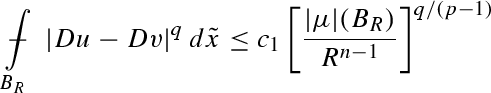(76)

and therefore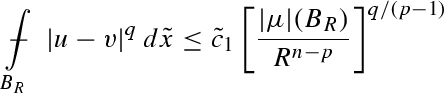(77)

hold for constants $c 1 , c ~ 1 ≡ c 1 , c ~ 1 ( n , p , ν , q )$, whenever

$0 < q < min p , n ( p - 1 ) n - 1 = : p m .$
(78)

### Proof

We restrict ourselves to the proof of (76), since this obviously implies (77) via Poincaré’s inequality. Moreover, we can restrict ourselves to prove (76) assuming $q > 1$, since the result for the remaining values would follow by Hölder’s inequality. Now, let us observe that we can always reduce to the situation when there exists $ξ > 1$ such that

$ξ q / ( p - q ) = q ∗ ,$
(79)

where $q ∗$ is the Sobolev conjugate of $q$. Indeed, when $p ≤ n$ then $p m = n ( p - 1 ) / ( n - 1 )$ and therefore $q < p m$ implies that $q / ( p - q ) < q ∗ = n q / ( n - q )$, so that (79) follows for the corresponding choice of $ξ$. When instead $p > n$ then we first observe that we can prove (76) in the case $n ≤ q < p$, since (76) for lower values of $q$ would then follow by Hölder’s inequality. Now, since by definition of Sobolev embedding exponent we can take $q ∗$ as large as we please, we again find a number $ξ > 1$ for which (79) holds. We then plan to apply Lemma 2 with this choice of $ξ$ and with the following one of $h$: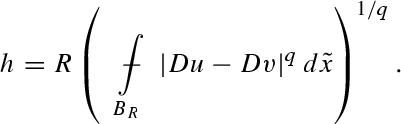Notice we can always assume that $h > 0$, otherwise the assertion of Lemma 2 trivializes. By using the definitions in the last two displays, inequalities (73) and (75), and finally Sobolev embedding theorem, we then have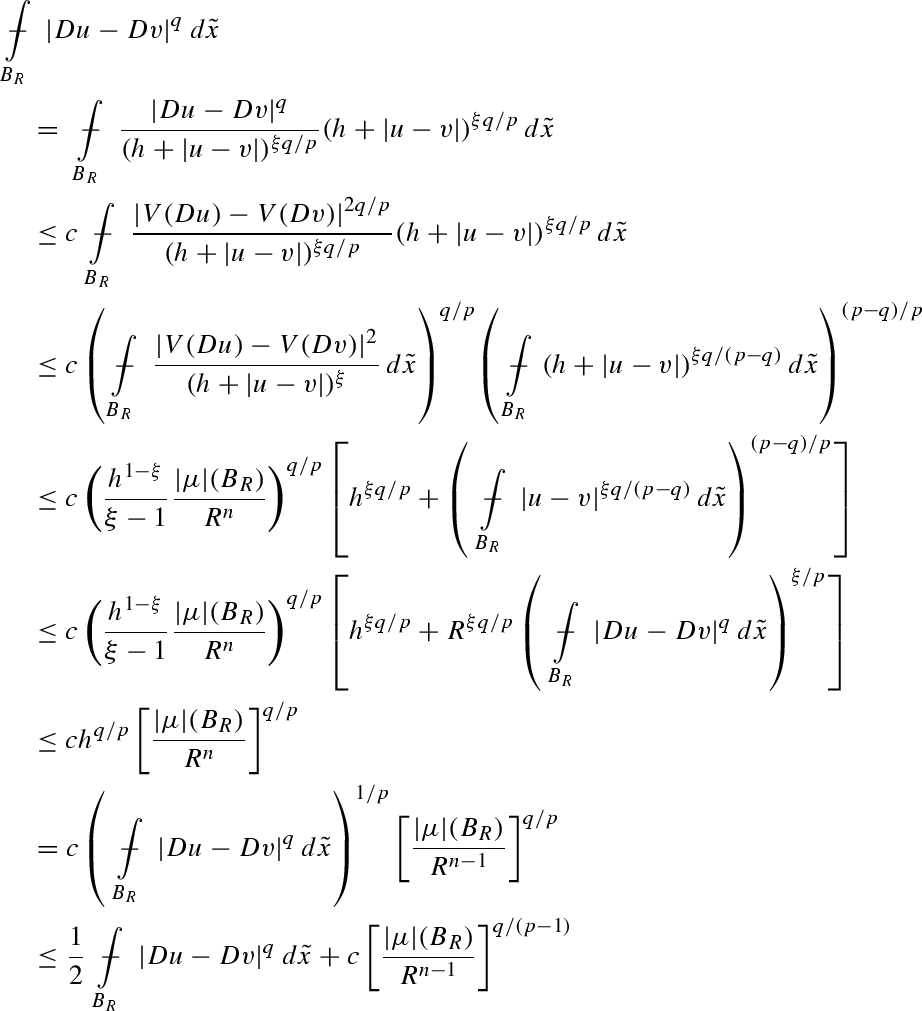so that (76) follows. $□$

### Remark 4

By examining the arguments of the previous lemma it is possible to see that the constant $c 1$ appearing in (76) shows the following natural asymptotic for $q → p m$:

$c 1 = c ( n , p , ν ) 1 p m - q q / ( p - 1 ) ,$
(80)

where $c$ is a constant that remains bounded whenever $p$ varies in a compact subset of $( 1 , ∞ )$; see (79) and the constant appearing in Sobolev–Morrey embedding theorem. The asymptotic in (80) is typical in situations when a borderline estimate in Lebesgue spaces fails, being replaced by an estimate in Marcinkiewicz spaces; recall (58).

## A sequence of comparison estimates

Given a number $δ 1 ∈ ( 0 , 1 / 4 )$ and a ball $B ( x , r ) ⊂ Ω$, we define the sequence of shrinking balls

$B j : = B ( x , r j ) , r j = δ 1 j r ,$
(81)

whenever $j ≥ 0$ is an integer. The related comparison solutions $v j ∈ u + W 0 1 , p ( B j )$ are as in (70), that is

$div a ( x , D v j ) = 0 in B j v j = u on ∂ B j .$
(82)

Then we have

### Lemma 3

Let $u ∈ W 1 , p ( Ω )$ be a solution to (17) under assumptions (18), and let $v j - 1 , v j$ be as in (82) with $j ≥ 1$. Suppose further that

$| μ | ( B j - 1 ) λ p - 1 r j - 1 n - 1 ≤ 1$
(83)

and that the bounds

$λ A ≤ | D v j - 1 | ≤ A λ in B j$
(84)

hold for constants $A ≥ 1$ and $λ > 0$. Then there exists a constant $c 2$ depending only on $n , p , ν , δ 1 , A$ such that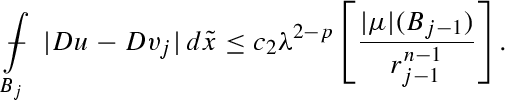(85)

### Proof

We start fixing the following quantities:

$γ : = 1 4 ( p - 1 ) ( n + 1 ) and ξ : = 1 + 2 γ ,$
(86)

in view of the application of Lemmas 1 and 2, that we shall use with exponents $q$ such that

$0 < q ≤ ξ ( p - 1 ) = p - 1 + 1 2 ( n + 1 ) .$

This in particular fixes the constant $c 1$ from Lemma 2. We also set

$v ¯ j - 1 : = v j - 1 λ , v ¯ j : = v j λ .$
(87)

In the rest of the proof constants denoted by $c$ will only depend on $n , p , ν , δ 1 , A$ and will in general vary from line to line, as usual. We start estimating the term on the left in (85) with the aid of (84) as follows: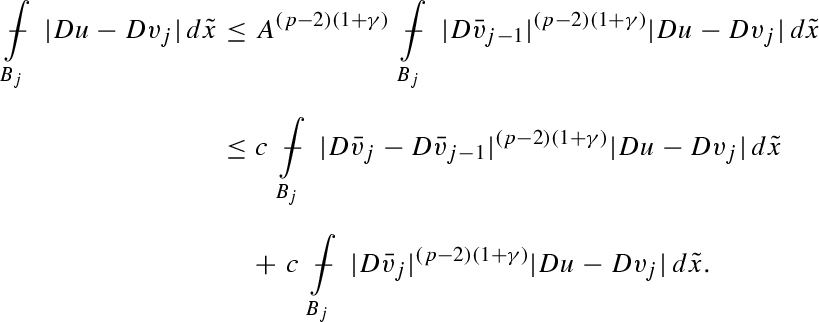(88)

To continue, we estimate the second-last integral appearing in the previous display. For this let us preliminary note that, by using (76) and recalling that $r j = δ 1 r j - 1$, the following estimate holds indeed for the same range of exponents $q$ working in (76):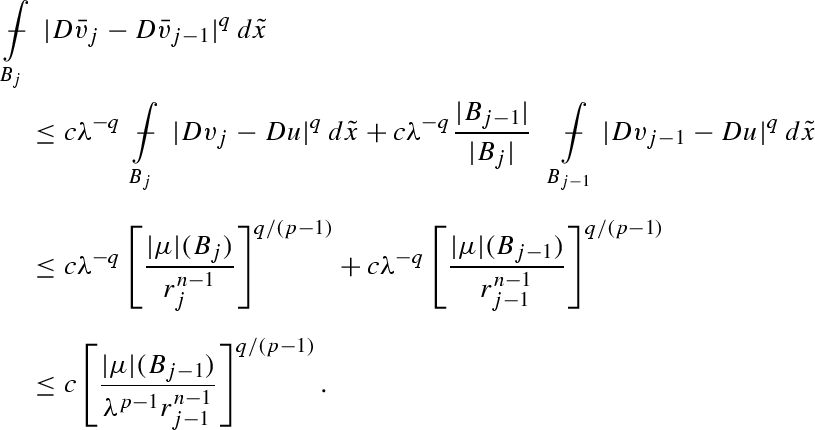(89)

Then, appealing to Hölder’s inequality together with (76), gives us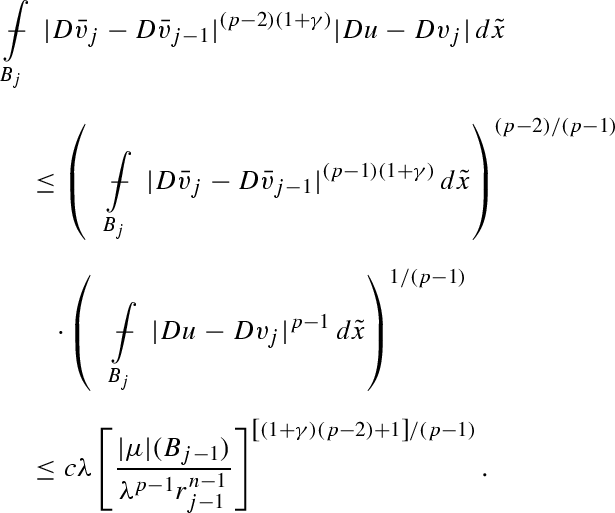(90)

But now, as

$( 1 + γ ) ( p - 2 ) + 1 p - 1 > 1$
(91)

then (83) implies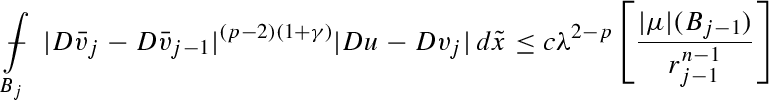with $c ≡ c ( n , p , ν , δ 1 )$. Combining this last estimate with (88) gives us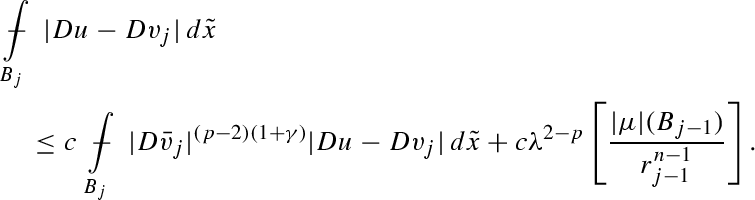(92)

It remains to estimate the first term on the right hand side in the above display. Applying Hölder’s inequality, together with (72) and (75), and recalling that $ξ = 1 + 2 γ$, we obtain for any $h > 0$ that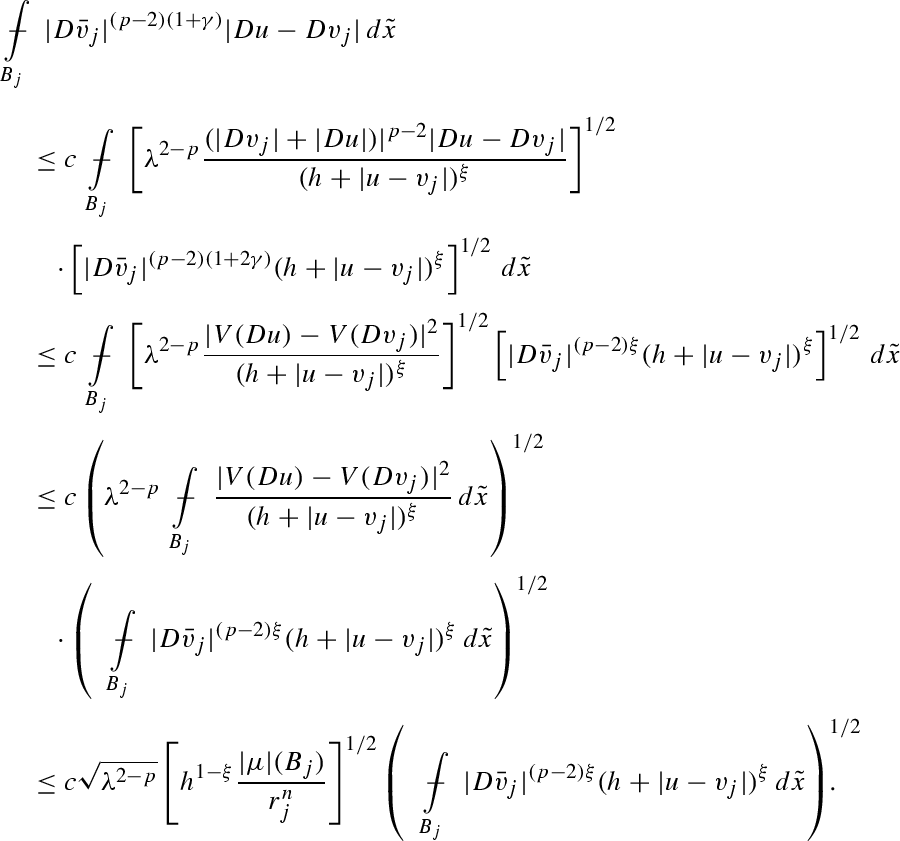(93)

Now we choose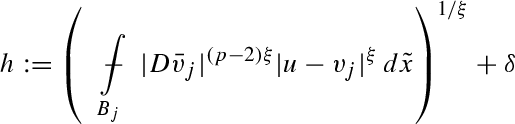(94)

for some small positive $δ ∈ ( 0 , 1 )$ to get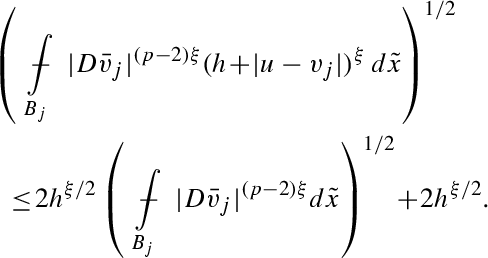Notice that the presence in (94) of the parameter $δ$ is aimed at guaranteeing that $h$ is positive; we shall eventually let $δ → 0$ at the end of the proof. In the above display, we use (89) and (84) to gain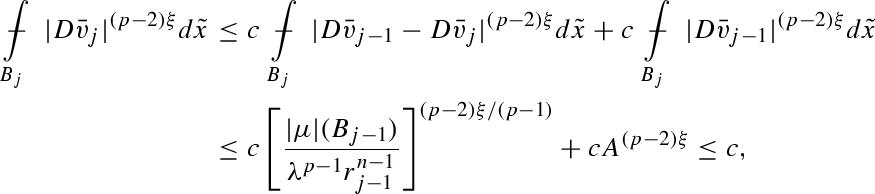so that we conclude with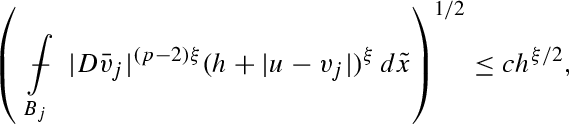where the constant $c$ ultimately depends only on $n , p , ν , δ 1 , A$. Plugging the last estimate in (93) now yields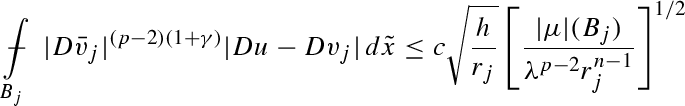while applying Young’s inequality and recalling again that $r j = δ 1 r j - 1$ gives(95)

whenever $ε ∈ ( 0 , 1 )$, where $c ≡ c ( n , p , ν , δ 1 , A )$ is in particular independent of $ε$. It finally remains to estimate $h$, which has been defined in (94). We have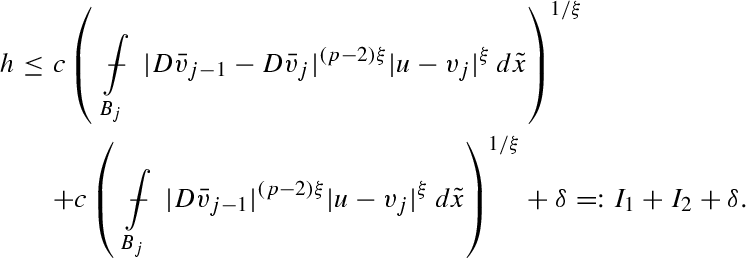(96)

Using (76) and (89) to estimate $I 1$ leads to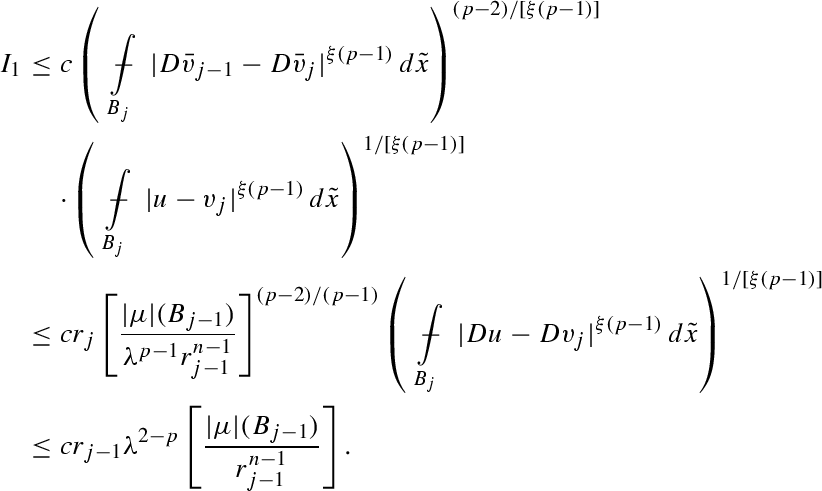(97)

In turn we bound $I 2$ appealing to (76) and using (84) repeatedly as follows:Similarly to (90) we find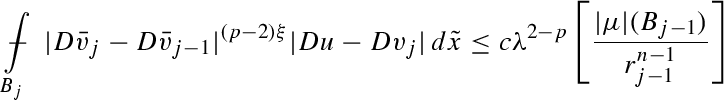so that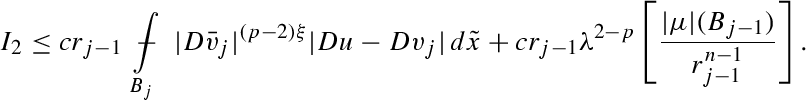Combining this last estimate with (97) and eventually with (96) yields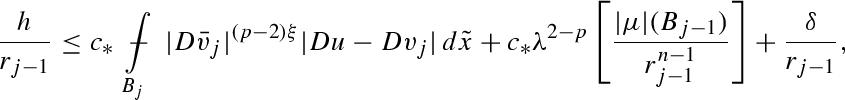where $c ∗$ depends only on $n , p , ν , L , A , δ 1$. Plugging the inequality in the above display in (95), choosing $ε = 1 / ( 2 c ∗ )$ and reabsorbing terms leads to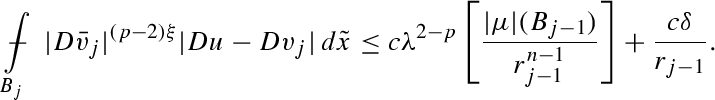Now (85) follows using this last inequality in combination with the one in display (92), and finally letting $δ → 0$. $□$

## Proof of Theorems 8 and 10

The proof of Theorem 10 falls in six steps, going through Sects. 12.112.6 respectively. It requires the use of several different tools, and ultimately relies on a delicate iteration technique. Finally, in Sect. 12.7, we shall briefly show how to get Theorem 8 from Theorem 10.

In the following, given a ball $B ⊂ Ω$, we define the excess of a vector field $f ∈ L 1 ( B , R k )$ over $B$ as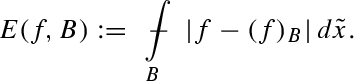This functional, roughly speaking, provides an integral measure of the oscillations of the vector field $f$ in the ball $B$. An elementary property of the excess is given by the following inequality: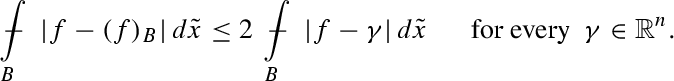(98)

We remark that here and in the following we shall use the comparison estimates presented in Sects. 10 and 11 that in fact applies in particular to the case of the solutions considered in Theorem 10.

### A density property of $a$-harmonic functions

In this section we shall consider a solution $v ∈ W loc 1 , p ( B ) ∩ W 1 , 1 ( B )$ to the equation

$div a ( D v ) = 0 in B ,$
(99)

where $B ⊂ R n$ is a given ball. We here restate in a suitable form the basic regularity properties a priori estimates for solutions to equation in (99); proofs can be for instance found in [18, 21, 51, 55]. The first is the classical gradient $L ∞ - L 1$ bound(100)

which is valid whenever $γ ∈ ( 0 , 1 )$ and for constant $c l$ depending only on $n , p , ν , L$. Next, we restate in suitable way the gradient Hölder continuity estimate included in display (35); more precisely, we select $β ≡ β ( n , p , ν , L ) ∈ ( 0 , α M )$ such that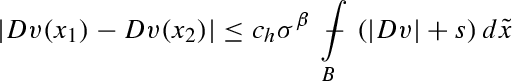(101)

holds whenever $σ B ⊂ B$ is a ball concentric to $B$ with $σ ∈ ( 0 , 1 / 2 )$, such that $x 1 , x 2 ∈ σ B$, where again $c h ≥ 1$ depends only on $n , p , ν , L$. Based on the previous regularity estimates, we can prove the following result, that roughly tells that something that happens in average, happens actually everywhere, and in a precisely quantifiable way.

### Proposition 2

(Density improvement) Let $v ∈ W loc 1 , p ( B ) ∩ W 1 , 1 ( B )$ be a solution to (99) such that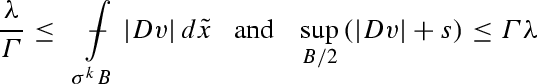(102)

hold for some integer $k ≥ 1$ and for numbers $Γ ≥ 1$, $λ > 0$ and $σ ∈ ( 0 , 1 / 4 )$ such that

$0 < σ ≤ 1 4 c h Γ 2 1 / β ,$
(103)

where $β$ and $c h$ are the constants appearing in (101). Then

$λ 4 Γ ≤ | D v | holds in σ B .$

### Proof

The first inequality in (102) implies that there exists a point $x 0 ∈ σ k B$ such that

$| D v ( x 0 ) | > λ 2 Γ .$

On the other hand, as (101) holds, the second inequality in (102) gives

$| D v ( x ) - D v ( x 0 ) | ≤ c h Γ λ σ β$

whenever $x ∈ σ B$, the last two inequalities and (103) then give

$| D v ( x ) | ≥ | D v ( x 0 ) | - | D v ( x ) - D v ( x 0 ) | ≥ λ 2 Γ - λ 4 Γ = λ 4 Γ$

for all $x ∈ σ B$. $□$

Finally, we recall two decay estimates that hold for the excess functional of the gradient and of the solutions. They are again related to the Hölder continuity of the gradient of solutions to (99) and of the solutions themselves, in the case we are considering homogeneous equations with measurable coefficients of the type

$div a ( x , D v ) = 0 in B .$
(104)

We now connect two basic regularity results for solutions to (99) and (104), respectively. They are bound to provide

### Theorem 17

[19, 21, 47] Let $v ∈ W loc 1 , p ( B ) ∩ W 1 , 1 ( B )$ be a weak solution to (99) under the assumptions (16). There exist constants $β ∈ ( 0 , 1 ]$ and $c d ≥ 1$, both depending only on $n , p , ν , L$, such that the estimate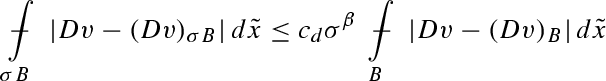(105)

holds whenever $σ B ⊂ B$ are concentric balls.

### Remark 5

The exponent $β$ appearing in (105) can be chosen arbitrarily close to the exponent $α M$ appearing in (34). As a matter of fact, estimate (35) is in fact obtained as a corollary of the one in (105) via the standard Campanato’s integral characterisation of Hölder continuous functions . The exponent $α M$ considered in Theorem 9 is indeed nothing but the sup of the numbers $β$ considerable in Theorem 17. Explicit, though not optimal, estimates on these numbers via the structure parameters $n , p , ν , L$, are retrievable tracking the dependence of the constants in the proofs in [19, 21, 47].

### Theorem 18

Let $v ∈ W loc 1 , p ( B ) ∩ W 1 , 1 ( B )$ be a weak solution to (104) under the assumptions (18), and where the vector field $a : Ω × R n → R n$ has measurable dependence on $x$. There exist constants $β ~ ∈ ( 0 , 1 ]$ and $c ~ d ≥ 1$, both depending only on $n , p , ν , L$, such that the estimate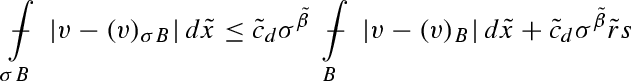(106)

holds whenever $σ B ⊂ B$ are concentric balls, where $r ~$ is the radius of $B$.

### Proof

The proof combines a few well-known facts in the regularity theory of the equation considered. Let us recall the following Caccioppoli type inequality, that states there exists a constant $c ≡ c ( n , p , ν , L )$ such that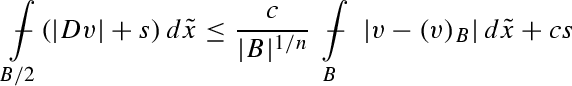(107)

holds; see [40, Proposition 4.1]. On the one hand, the following gradient decay estimate holds whenever $σ ∈ ( 0 , 1 / 2 ]$ (see for instance [55, Lemma 3.3]):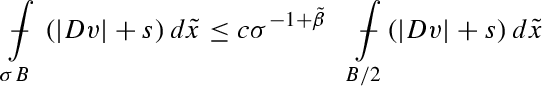(108)

where $β ~ < α m$ and $c ≡ c ( n , p , ν , L , β ~ )$. Both the inequalities in the last displays are typical in the literature (see for instance [25, Chapter 7]) when using $L p$-norms instead of the $L 1$-ones. Combining them then yields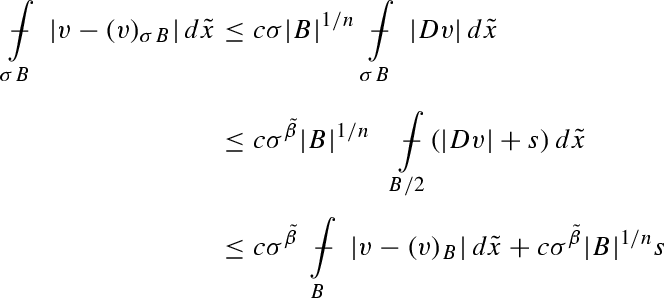that proves (107) in the case $σ ∈ ( 0 , 1 / 2 ]$. On the other hand, the remaining case $σ ∈ ( 1 / 2 , 1 ]$ is trivial by using (98). $□$

### Remark 6

Exactly as in Remark 5, the exponent $β ~$ appearing in Theorem 18 can be actually taken as close to $α m$—appearing in (30)–(31)—as we please. As a matter of fact estimates (31) and (106) are equivalent by noticing that if $v$ solves then so does $v - k$, whenever $k$ is a real number. Again, the exponent $α m$ used in Theorem 6 is actually defined as the sup of the numbers $β$ for which (106) works. Estimates for such numbers are available in terms on the structure parameters $n , p , ν , L$.

### Setting of the constants

In the following all the balls considered will be centered at the point $x ∈ Ω$, and we start form a ball $B R ≡ B ( x , R ) ⊂ Ω$ as in the statement of the theorem. We introduce $λ M$ as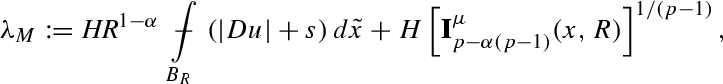(109)

and fix the constant $H ≥ 1$ in a few lines (see (114) below), in a way that makes it depending only on $n , p , ν , L$; we will then prove that

$M 1 - α , R ( D u ) ( x ) ≤ c λ M$
(110)

holds uniformly in $α ∈ [ 0 , 1 ]$, for yet another constant $c ≡ c ( n , p , ν , L )$. Clearly, we may assume without loss of generality that $λ M > 0$, otherwise there is nothing to prove. Let us now fix a few constants that will be relevant in the following; the constants $c l , c h , c d$ have been defined in (100), (101) and Theorem 17, respectively, while $c 1$ has been introduced in Lemma 2 (that here will be used with $q = 1$); all these constants depend only on $n , p , ν , L$. Again, $β ≡ β ( n , p , ν , L ) ∈ ( 0 , 1 )$ is the exponent appearing in (101) and (105). Then we fix

$δ 1 : = 1 10 8 n c l 2 c d c h 1 / β .$
(111)

It follows that also $δ 1$ depends only on $n , p , ν , L$. With such a choice of $δ 1$ we consider the balls in  (81), that is

$B j : = B ( x , r j ) , r j = δ 1 j r , r : = R / 2 ,$

and the related comparison solutions $v j ∈ u + W 0 1 , p ( B j )$ in (82), that is

$div a ( D v j ) = 0 in B j v j = u on ∂ B j .$
(112)

Again, with $δ 1$ now being fixed we determine the constant $c 2$ from Lemma 3 as follows:

$c 2 : = c 2 ( n , p , ν , δ 1 , A ) with A : = 10 4 n c l .$
(113)

The following inclusions hold for every $j ≥ 0$, and the will be used throughout the proof: $δ 1 B j = B j + 1 ⊂ B j ⊂ ( 1 / 4 ) B j ⊂ B j .$ We finally set

$H : = 10 8 c 1 c 2 c d δ 1 - 5 n$
(114)

and again notice that $H$ only depends on $n , p , ν , L$. Now, let us set

$κ : = min 1 , δ 1 n - p + α ( p - 1 ) and r ~ j : = r j + r j + 1 2$

for $j ≥ 0$, and observe that

$I p - α ( p - 1 ) μ ( x , R ) = ∫ 0 2 r | μ | ( B ϱ ) ϱ n - p + α ( p - 1 ) d ϱ ϱ = ∑ j = 0 ∞ ∫ r ~ j r j | μ | ( B ϱ ) ϱ n - p + α ( p - 1 ) d ϱ ϱ + ∫ 8 r / 7 2 r | μ | ( B ϱ ) ϱ n - p + α ( p - 1 ) d ϱ ϱ + ∑ j = 0 ∞ ∫ r j + 1 r ~ j | μ | ( B ϱ ) ϱ n - p + α ( p - 1 ) d ϱ ϱ + ∫ r 8 r / 7 | μ | ( B ϱ ) ϱ n - p + α ( p - 1 ) d ϱ ϱ ≥ ∑ j = 0 ∞ ∫ r ~ j r j | μ | ( B ϱ ) ϱ n - p + α ( p - 1 ) d ϱ ϱ + ∫ 8 r / 7 2 r | μ | ( B ϱ ) ϱ n - p + α ( p - 1 ) d ϱ ϱ ≥ κ ∑ j = 0 ∞ | μ | ( B ¯ j + 1 ) r j + 1 n - p + α ( p - 1 ) ∫ r ~ j r j d ϱ ϱ + | μ | ( B ¯ 0 ) 2 n - 1 r n - p + α ( p - 1 ) ∫ 8 r / 7 2 r d ϱ ϱ ≥ δ 1 n - 1 log 2 1 + δ 1 ∑ j = 0 ∞ |$